• 采用源极耦合动态多值电流模电路，利用条件和算法，设计实现了基于四值逻辑的8-bit加法器。利用HSPICE软件，在0.18μmCMOS工艺下，电源电压为1.8V，时钟频率为100MHz的条件下，进行了仿真。仿真结果表明，所设计的...
• 加法器是最常用的运算结构，因此加法器的优化在集成电路的优化就显得极为重要，有两种优化方法。1、逻辑层优化，重新安排一个布尔方程以得到一个速度较快或者说面积较小的电路。2、电路层优化，改变晶体管的尺寸或者...
基础知识
加法器是最常用的运算结构，因此加法器的优化在集成电路的优化就显得极为重要，有两种优化方法。1、逻辑层优化，重新安排一个布尔方程以得到一个速度较快或者说面积较小的电路。2、电路层优化，改变晶体管的尺寸或者电路的拓扑结构。对于一个二进制加法器来说，由二进制全加器的真值表可以得到他的布尔表达式。 简单全加器结构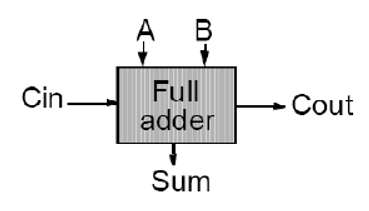二进制全加器真值表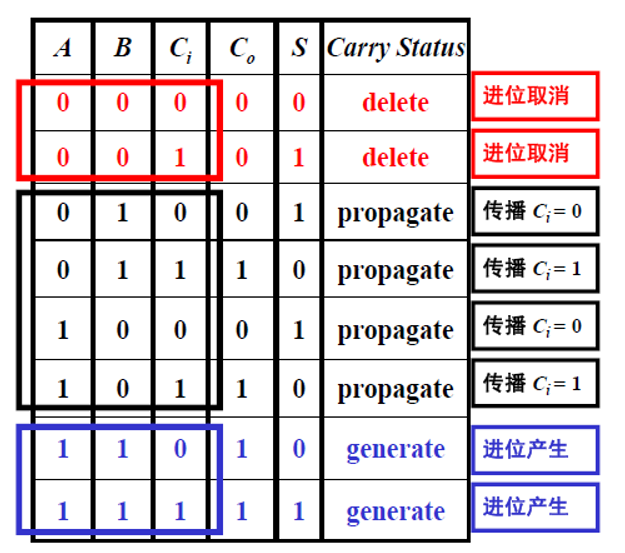这里说一下carry status,简单来说三个状态的区分取决于A、B的值。 和（S）和进位（C0）布尔表达式：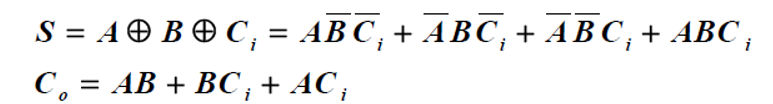中间信号可以观察出来：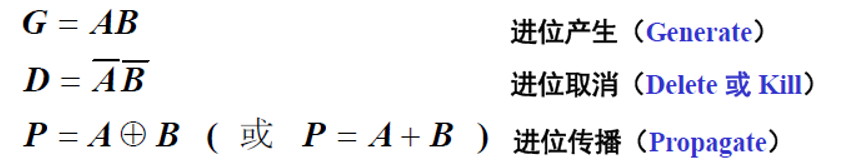逐位加法器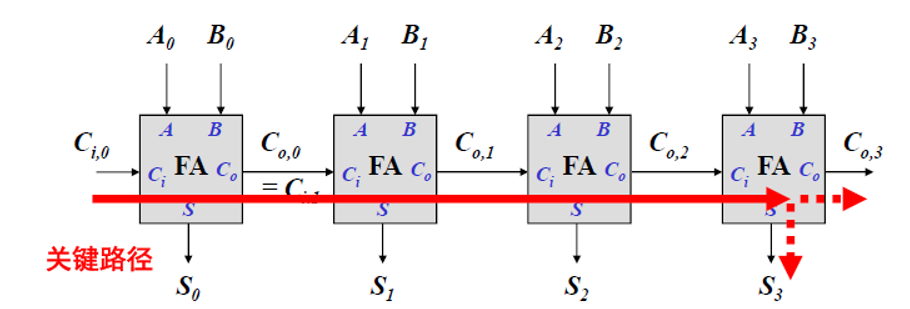上图是一个4为的逐位加法器，其中的关键路径就是指延时最长的路径，通常用关键路径来决定着一个电路的性能。因此有：t(adder)=(N-1)t(carry)+t(sum) 在实际复杂电路中，加法器的位数往往是64位或者128位，因此优化**t(carry)**更为重要。
全加器的电路设计
1、静态加法器 2、镜像加法器 3、传输门型加法器 4、曼彻斯特进位链加法器（有静态和动态之分）
静态互补CMOS加法器电路
原理：利用逻辑方程直接转变成CMOS电路，进行某些逻辑变换。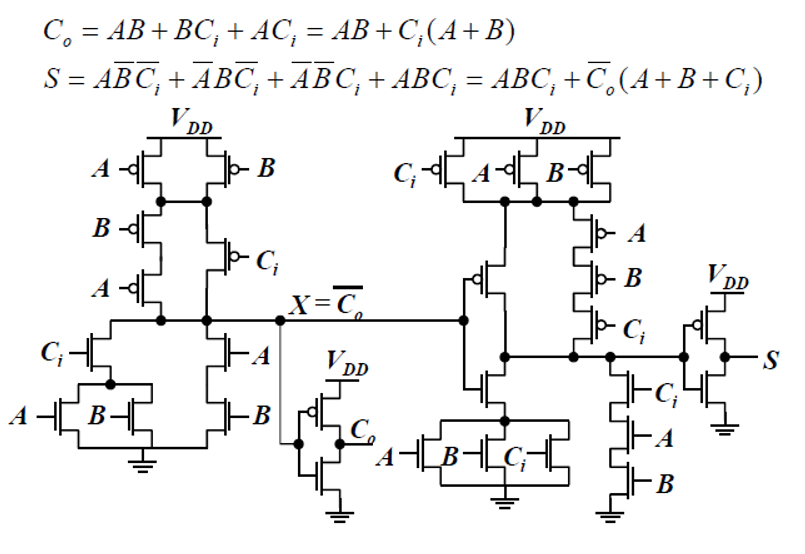这个电路总共需要28个晶体管，面积和速度都比较慢，产生这种现象的原因有以下几点：
再进位产生于和产生电路之间堆叠着许多的PMOS管 。C0信号的本征负载电容比较大。
对电路进行初步的优化：去除偶数级的反相器。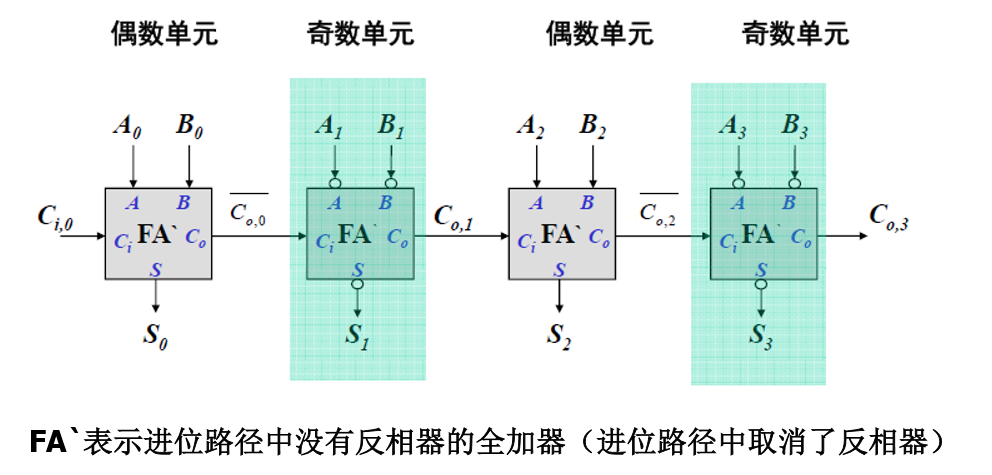优化原理：把一个全加器的所有输入反向，则它的说有所有输出也会反相。
镜像CMOS加法器设计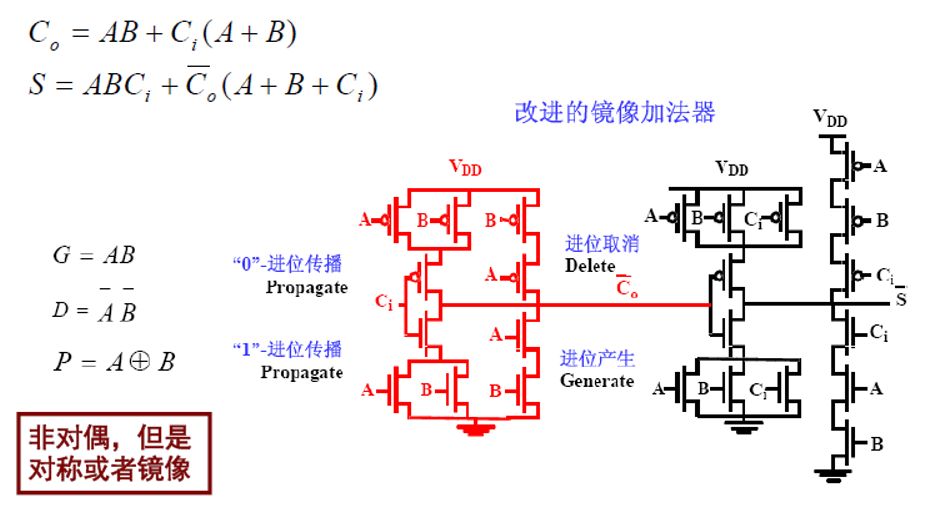优化原理：取消了进位反相门，PDN和PUN不再是对偶网络。 优化分析：
该全加器单元需要24个晶体管，NMOS和PMOS链完全对称，在产生的进位的电路的部分，最多有两个管子串联。
传输门型加法器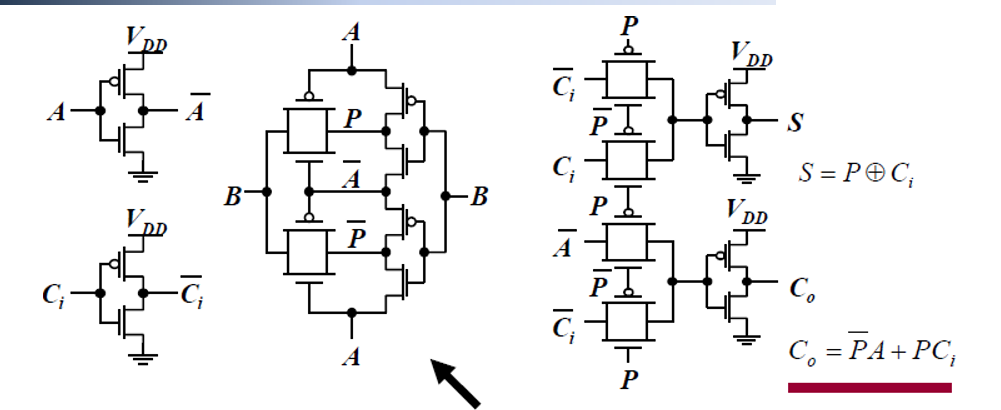共24个管子，最大的特点是它的和与进位输出具有相似的延时。
曼彻斯特进位链加法器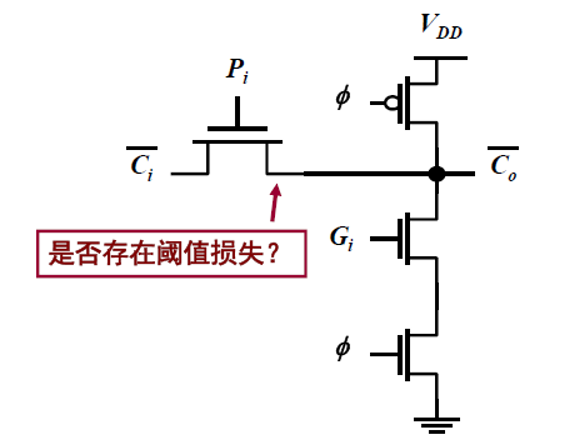动态实现，只用到进位传播和进位产生。 特点：
采用动态逻辑降低复杂性和加快速度；预充电时所有中间节点被预充至VDD ，求值时有条件放电；进位链传输管只用N管，节点电容很小，为四个扩散电容；进位链的分布RC本质使传播延时与位数N的平方成正比，因此有必要插入缓冲器；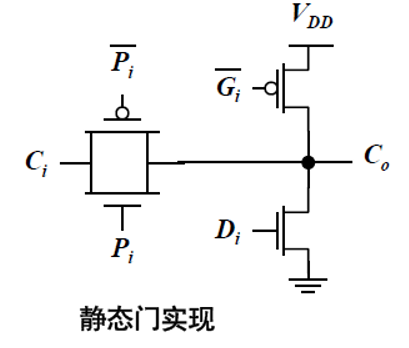静态实现，采用进位传播、进位消除、进位产生。 特点：
不需要时钟、预充电，可异步工作，一旦给出运算数a，b进位链马上工作，由此可提高速度。采用CMOS , 可降低功耗。如进位链较长时，应在Ci，Ci-1… 回路上插入缓冲级。
展开全文数字集成电路
• ## 先行进位加法器

千次阅读 2011-09-30 11:23:00
串行进位加法器需要一级一级的进位，进位延迟很大。先行进位加法器（也叫超前进位加法器）可以有效的减少进位延迟。 设二进制加法器的第i位输入为Xi, Yi, 输出为Si, 进位输入为Ci，进位输出为Ci+1 则有 Si = Xi...

串行进位加法器需要一级一级的进位，进位延迟很大。先行进位加法器（也叫超前进位加法器）可以有效的减少进位延迟。
设二进制加法器的第i位输入为Xi, Yi, 输出为Si, 进位输入为Ci，进位输出为Ci+1

则有
Si = Xi⊕Yi⊕Ci

Ci+1  = Xi·Yi + Xi·Ci + Yi·Ci = Xi·Yi + (Xi + Yi)·Ci
令Gi = Xi·Yi,     Pi = Xi+Yi
则Ci+1  = Gi + Pi·Ci
当Xi和Yi都为1时，Gi = 1，产生进位Ci+1 = 1
当Xi和Yi有一个为1时，Pi = 1，传递进位Ci+1 = Ci
因此Gi 定义为进位产生信号，Pi定义为进位传递信号。Gi的优先级比Pi高，也就是说：当Gi = 1时（当然此时也有 Pi = 1 ），无条件产生进位，而不管Ci是多少;
当Gi=0而Pi=1时，进位输出为Ci, 跟Ci之前的逻辑有关。
下面推导4位超前进位加法器。设4位加数和被加数为A 和 B，进位输入为Cin, 进位输出为Cout, 对于第i位的进位产生Gi = Ai·Bi , 进位传递 Pi=Ai+Bi , i=0,1,2,3
于是这各级进位输出，递归的展开Ci ，有：
C0 = Cin
C1=G0 + P0·C0
C2=G1 + P1·C1 = G1 + P1·（G0 + P0·C0）=G1 + P1·G0 + P1·P0 ▪C0
C3=G2 + P2·C2 = G2 + P2·G1 + P2·P1·G0 + P2·P1·P0·C0
C4=G3 + P3·C3 = G3 + P3·G2 + P3·P2·G1 + P3·P2·P1·G0 + P3·P2·P1·P0·C0
Cout=C4
由此可以看出，各级的进位彼此独立产生，只与输入数据和Cin有关，将各级间的进位级联传播给去掉了，因此减小了进位产生的延迟。
每个等式与只有三级延迟的电路对应，第一级延迟对应进位产生信号和进位传递信号，后两级延迟对应上面的积之和，这可由Synplify综合后的RTL电路看到。
同时由真值表可以简单的得出第i位的和为：
Si = Xi⊕Yi⊕Ci = (Xi·Yi)⊕(Xi+Yi)⊕Ci = Gi⊕Pi⊕Ci
根据上面的式子便可以设计出超前进位加法器。按照《数字系统设计与Verilog HDL》中的方法，用Verilog编写了4位超前进位加法器，代码如下：

module add_ahead(a,b,sum,cin,cout    );input [3:0] a,b;input cin;output [3:0] sum;output cout;wire c1,c2,c3;//各级进位输出wire [3:0] g,p;//产生第 0 位的本位值和进位输出assign g= a& b;assign p= a| b;assign sum= g^p^cin;assign c1=g|(p&cin);//产生第 1 位的本位值和进位输出assign g= a& b;assign p= a| b;assign sum= g^p^c1;assign c2=g|(p&c1);//产生第 2 位的本位值和进位输出assign g= a& b;assign p= a| b;assign sum= g^p^c2;assign c3=g|(p&c2);//产生第 3 位（最高位）的本位值和进位输出assign g= a& b;assign p= a| b;assign sum= g^p^c3;assign cout=g|(p&c3);endmodule

这段代码在实现c2,c3,cout的时候，仍然用了前一级的进位，我开始以为综合器会自动展开，但是用Synplify综合后发现，每一位的进位仍然与前一位的进位输出有关。于是将上面计算c2,c3,cout的代码用上面展开后的公式实现，如下：

assign c2=g|(p&g)|(p&p&cin);assign c3=g|(p&g)|(p&p&g)|(p&p&p&cin);assign cout=g|(p&g)|(p&p&g)|(p&p&p&g)|(p&p&p&p&cin)；

再用Synplify综合，发现c1,c2,c3,cout都只与初始进位和输出数据有关，各级进位间不存在进位延迟了。
修改后的程度用Modelsim仿真结果正确。

转载于:https://www.cnblogs.com/haigege/archive/2011/09/30/2196275.html
展开全文• 备战秋招-手撕代码篇 手撕代码verilog-任意整数分频 异步FIFO（二）——手撕代码 【老邱数电】半加器与全加器基础 单片机功能这么强，为什么还要...数字电路中全加器是什么原理，专用的加法器芯片有哪些 ...
前言
之前的文章《verilog学习:使用VCS仿真验证一个全加器》，示范了下全加器，这篇文章就展开说一下加法器。
参考的视频有： 【老邱数电】半加器与全加器基础 单片机功能这么强，为什么还要组合逻辑器件？ 数字电路中全加器是什么原理，专用的加法器芯片有哪些 0200410-104536-数字电路（1）-半加器和全加器的设计1
加法器分为1位数加法器和多位数加法器，1位数加法器又可以分为半加器和全加器。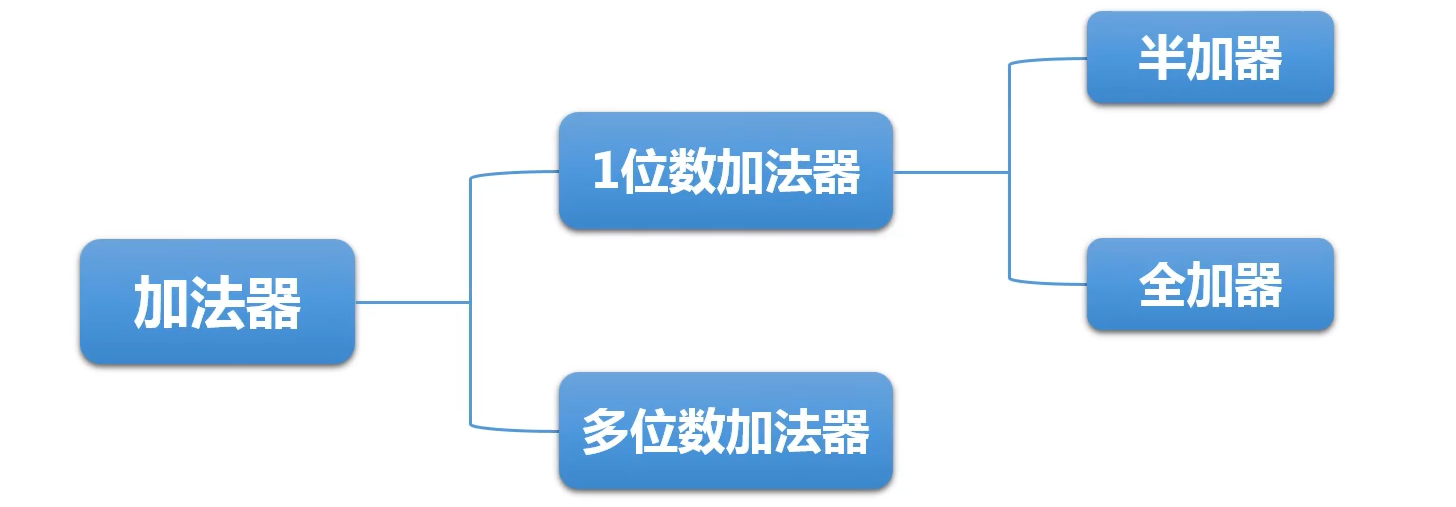半加：两个1位二进制数相加，不考虑来自低位的进位（2个二进制数相加）；全加：两个1位二进制数与来自低位的进位三者相加（3个二进制数相加）；半加器+半加法和全加法是算术运算电路中的基本单元，它们是完成1位二进制相加的一种组合逻辑电路。
不考虑低位的进位信号，将两个一位二进制数相加，只求本位和
二进制加法隐含条件：变量只取0和1；逢二进位；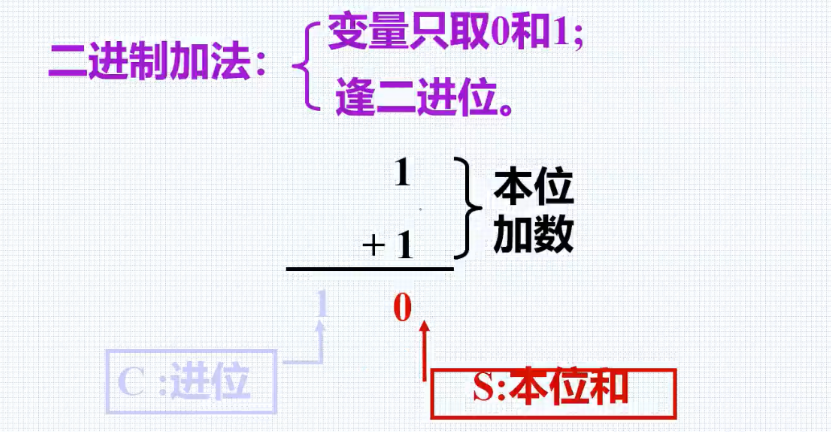真值表如下：
被加数A加数B和数S进位CO0000011010101101
由表中可以看见，这种加法没有考虑低位来的进位，所以称为半加。
跟据真值表，写出方程；根据表达式画出逻辑图：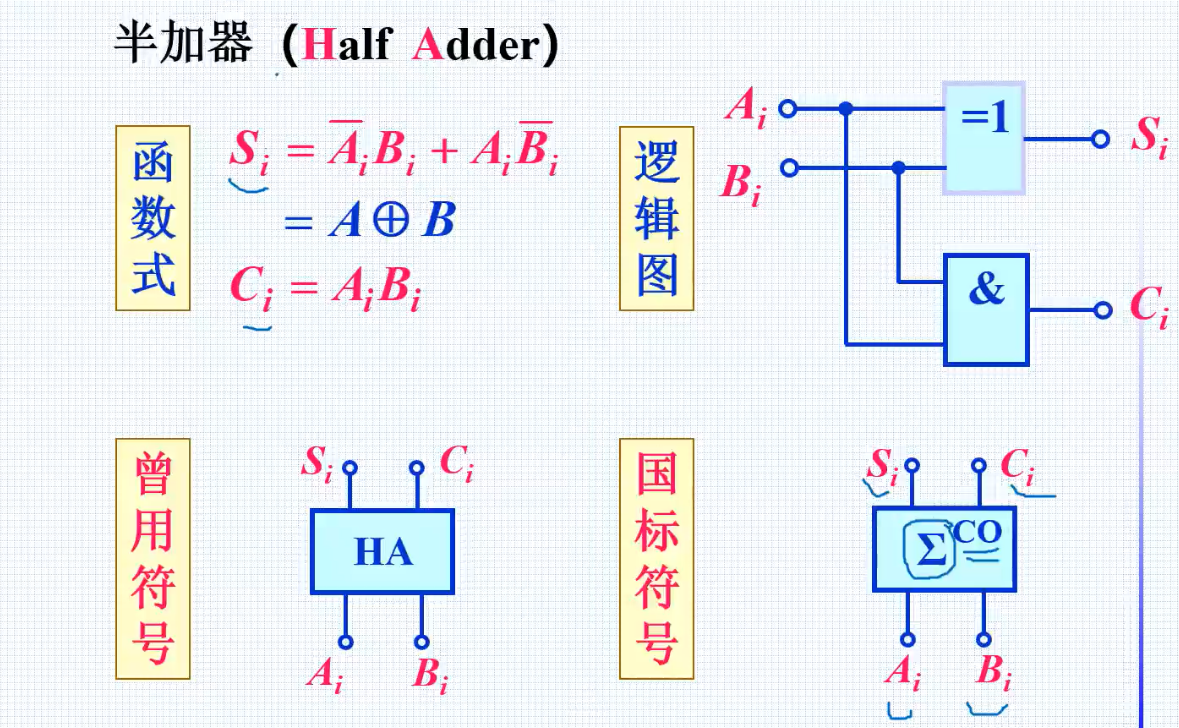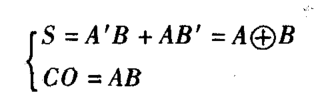1.1 半加器verilog设计代码
第一种写法：
module half_adder(
input a,             //第一个加数a
input b,             //第二个加数b
output sum,          //和
output cout          //位
);

assign sum=a ^ b;     //sum=a⊕b
assign cout=a & b;	  //cout=ab

endmodule

第二种写法：
module half_adder(cout,sum,a,b);
output   cout;
output   sum;
input    a,b;
wire     cout,sum  ;

//assign   {cout,sum}=a+b;
assign cout = a & b;
assign sum = a ^ b;

endmodule

这两种写法综合出来的电路图是一样的，如下：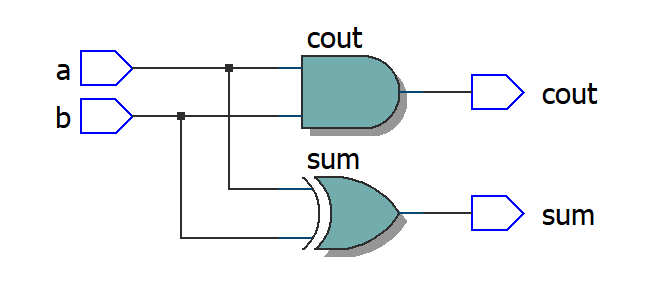第三种写法：
module half_adder(cout,sum,a,b);
output   cout;
output   sum;
input    a,b;
wire     cout,sum  ;

assign   {cout,sum}=a+b;
//assign cout = a & b;
//assign sum = a ^ b;

endmodule

第三种写法综合出来的电路图如下：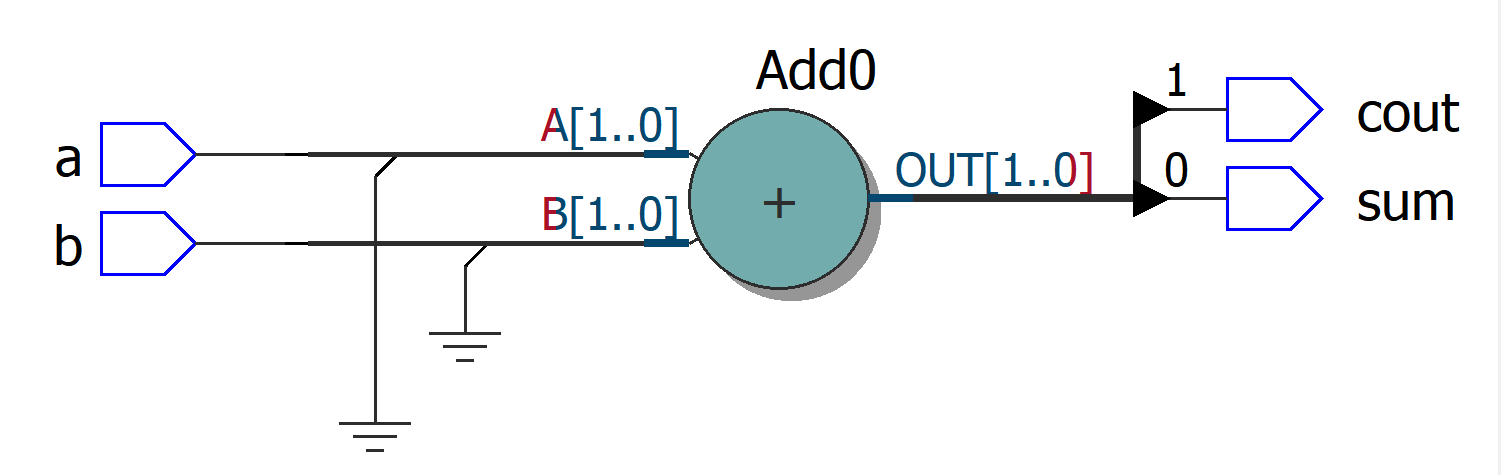1.2 测试文件testbench
timescale 1ns/10ps
reg    a,b;
wire   sum,cout;
integer i,j;

.sum  ( sum ),
.cout  ( cout),
.a     ( a  ),
.b     ( b  )
);

initial begin
a=0;b=0;
for(i=1;i<16;i=i+1)
#20   a=i;
end

initial begin
for(j=1;j<16;j=j+1)
#10   b=j;
end

initial begin
$monitor($time,,,"%d + %d ={%b,%d}",a,b,cout,sum);
#160  $finish; end endmodule  三种方式产生的波形文件都一样（注意，这里是1bit数相加，高位会溢出的）！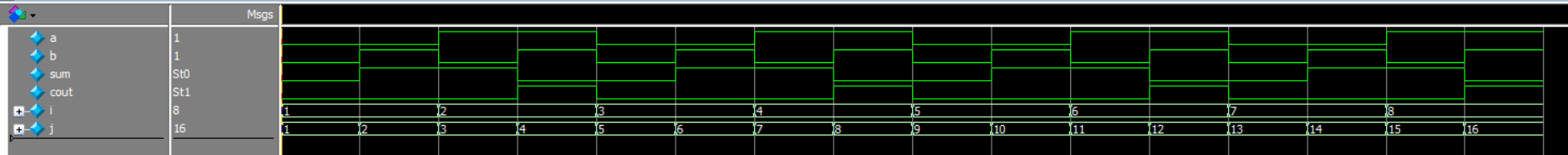2.全加器(Full Adder) 半加法器中，不考虑输入进位，在全加法器设计中将第i-1位的输出进位当 作第i位的输入。在将两个多位二进制数相加时，除了最低位以外，每一位都应该考虑来自低位的进位，即将两个对应位的加数和来自低位的进位3个数相加。这种运算称为全加，所用的电路称为全加器。两个1位二进制数相加，考虑低位进位 一位全加器的真值表如下图，其中Ai为被加数，Bi为加数，相邻低位来的进位数为Ci-1，输出本位和为Si。向相邻高位进位数为Ci 进位输入Ci-1被加数Ai加数Bi本位和Si进位输出Ci0000000110010100110110010101011100111111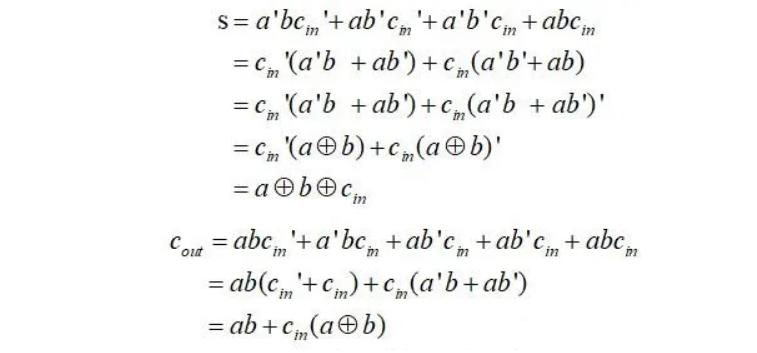一位全加器的逻辑表达式如下： Si=Ai⊕Bi⊕Ci-1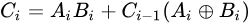电路图如下：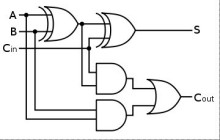2.1 全加器verilog设计代码 第一种写法： module full_add (a,b,cin,sum,cout); input a,b,cin; output sum,cout; reg sum,cout; always @(a or b or cin)begin sum = a ^ b ^ cin; cout = a & b |(cin&(a^b)); end endmodule  综合出来的电路：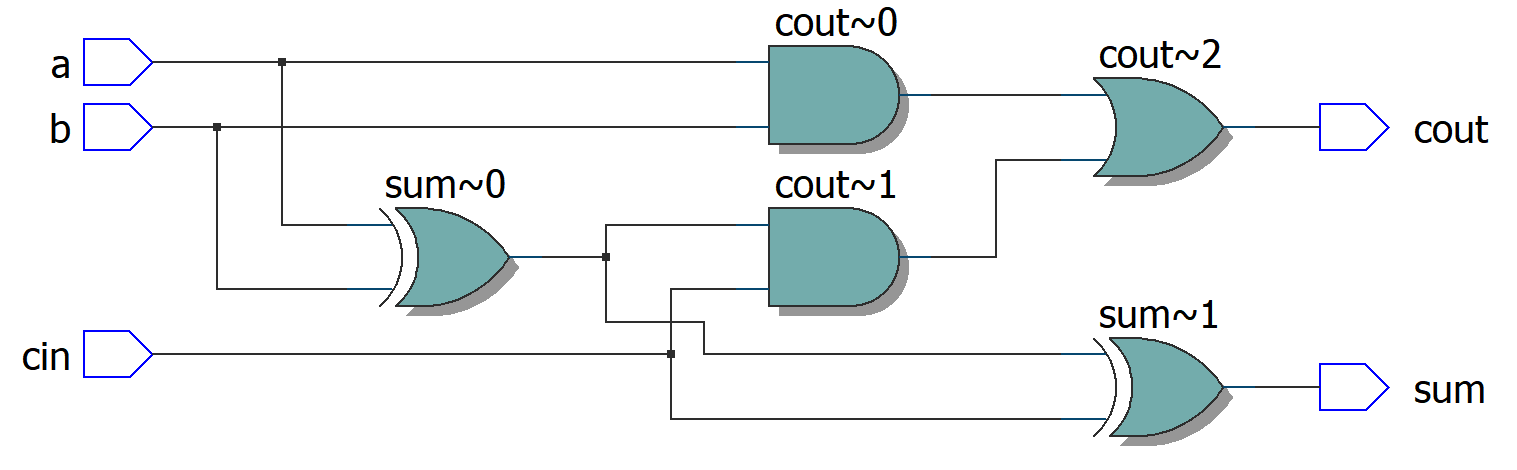第二种写法： module full_add (a,b,cin,sum,cout); input a,b,cin; output sum,cout; reg sum,cout; always @(a or b or cin)begin sum = a ^ b ^ cin; //cout = a & b |(cin&(a^b)); cout = (a & b)|(b & cin)|(a & cin); end endmodule  综合出来的电路：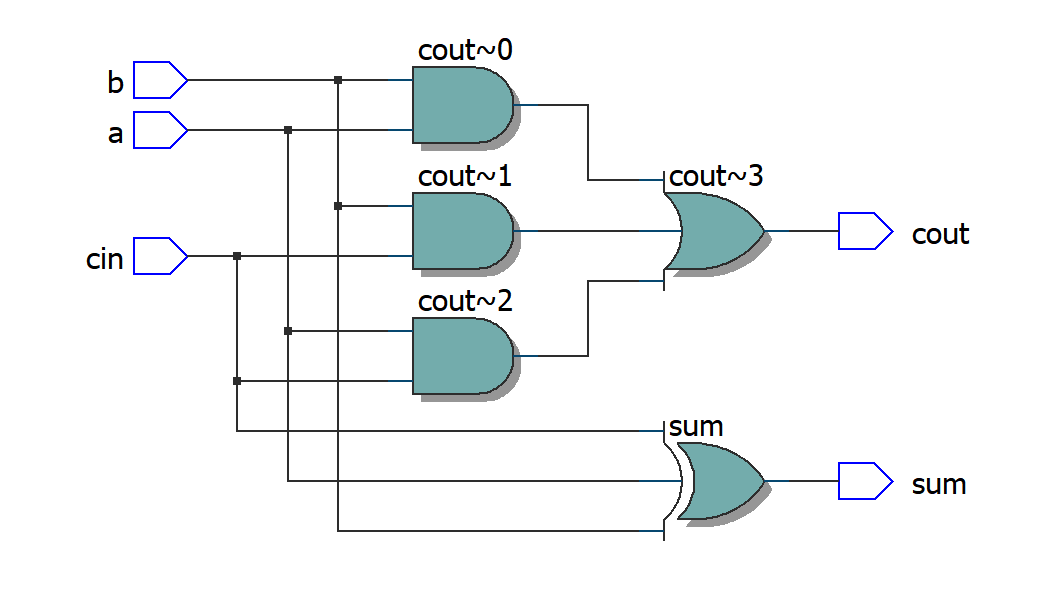第三种写法： module full_add (a,b,cin,sum,cout); input a,b,cin; output sum,cout; reg sum,cout; reg m1,m2,m3; always @(a or b or cin)begin {cout,sum} = a + b + cin; end endmodule  综合出来的电路：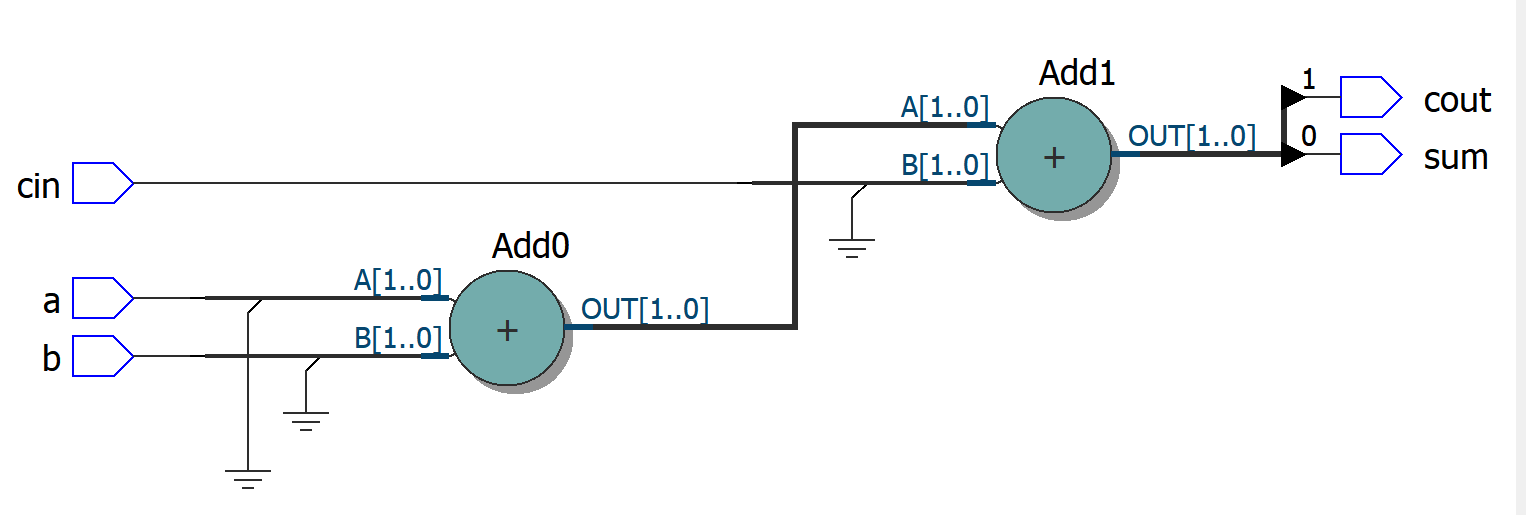2.2 测试文件testbench timescale 1ns/10ps //include "full_add.v" module full_add_testbench; reg a,b,cin; wire sum,cout; integer i,j,k; full_add full_adder_te( .sum ( sum ), .cout ( cout), .a ( a ), .b ( b ), .cin ( cin) ); initial begin a=0; for(i=1;i<16;i=i+1) #20 a=~a; end initial begin b=0; for(j=1;j<16;j=j+1) #10 b=~b; end initial begin cin=0; for(k=1;k<16;k=k+1) #5 cin=~cin; end initial begin$monitor($time,,,"%d + %d + %d ={%b,%d}",a,b,cin,cout,sum); #80$finish;
end

endmodule

这三种方法的仿真波形都一样，可对比：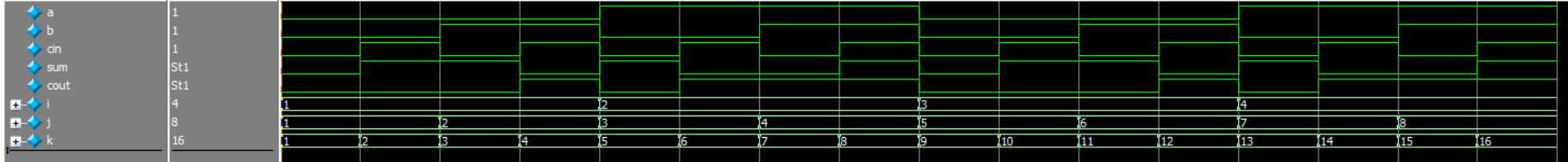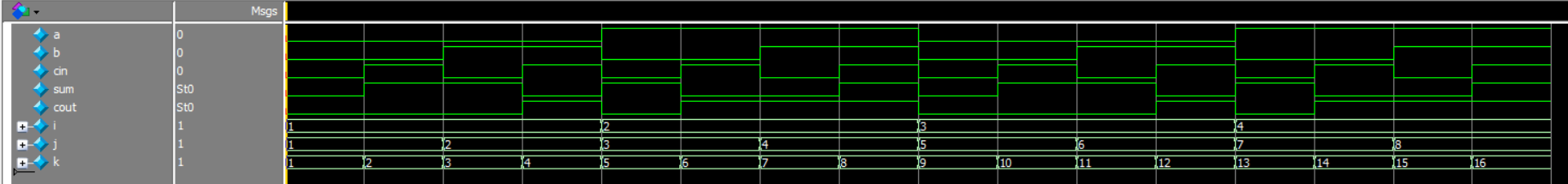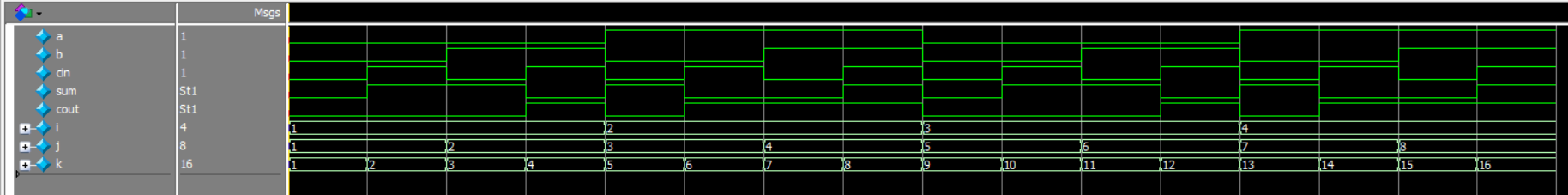3.其实还有很多种加法器，可深入了解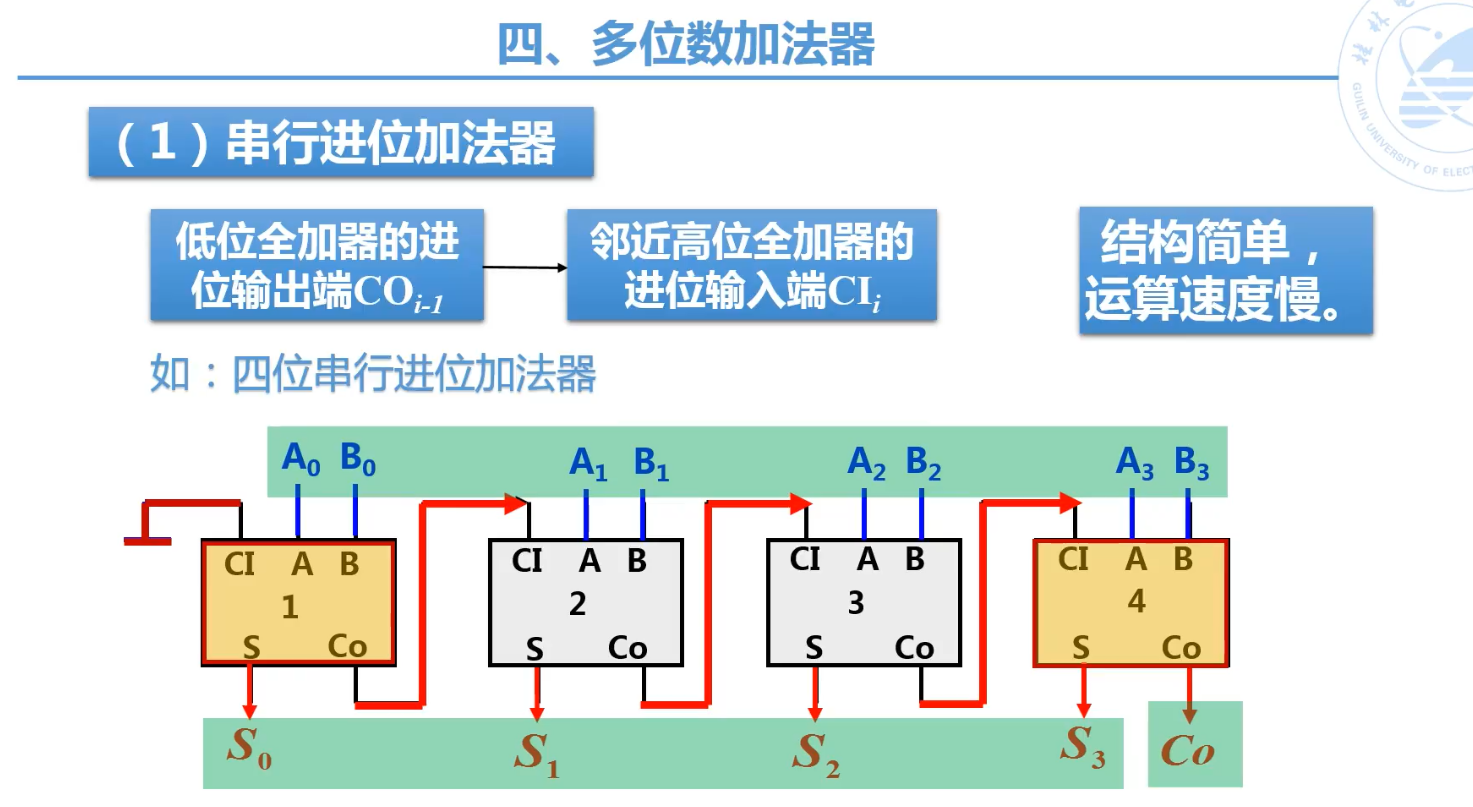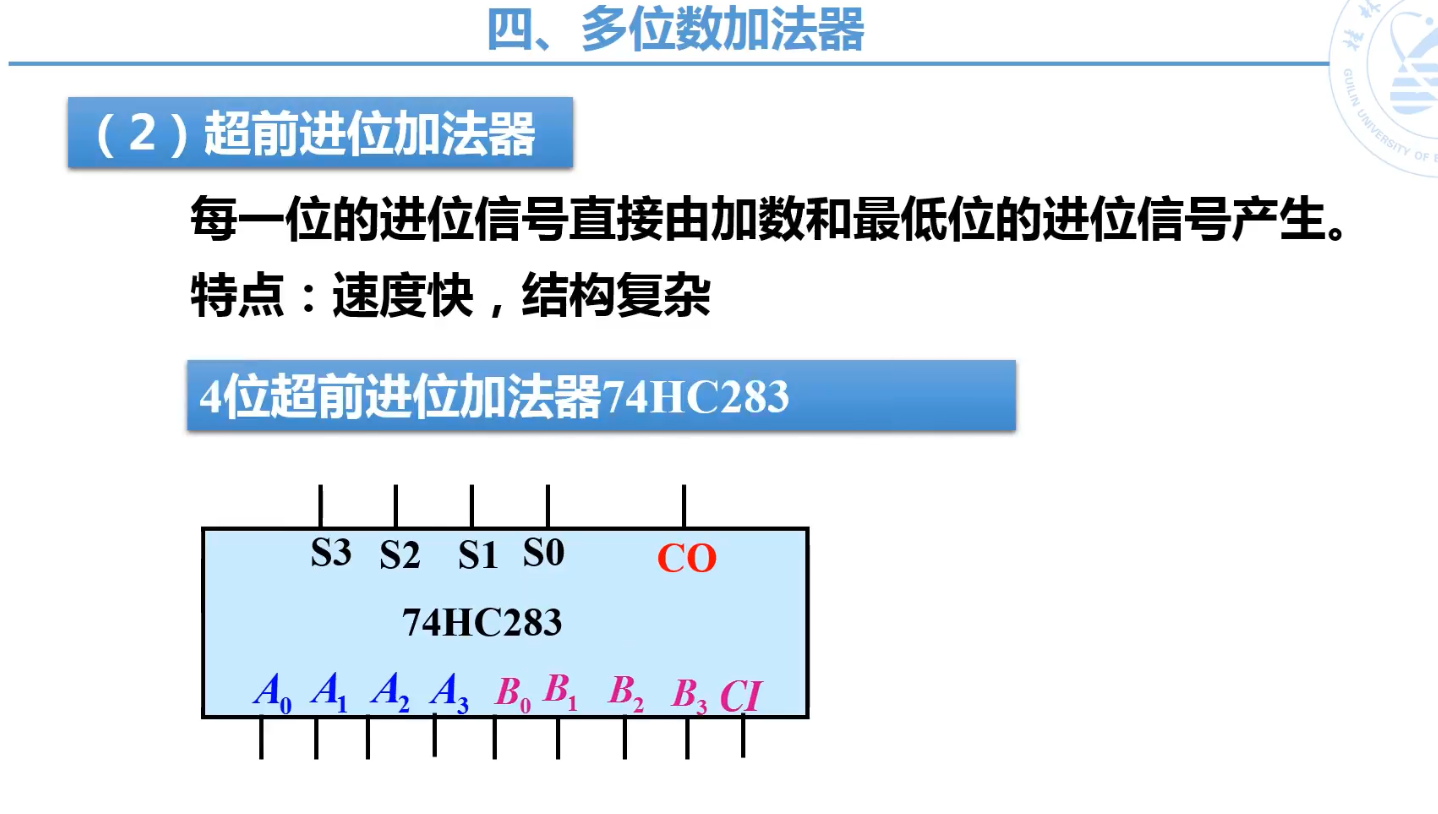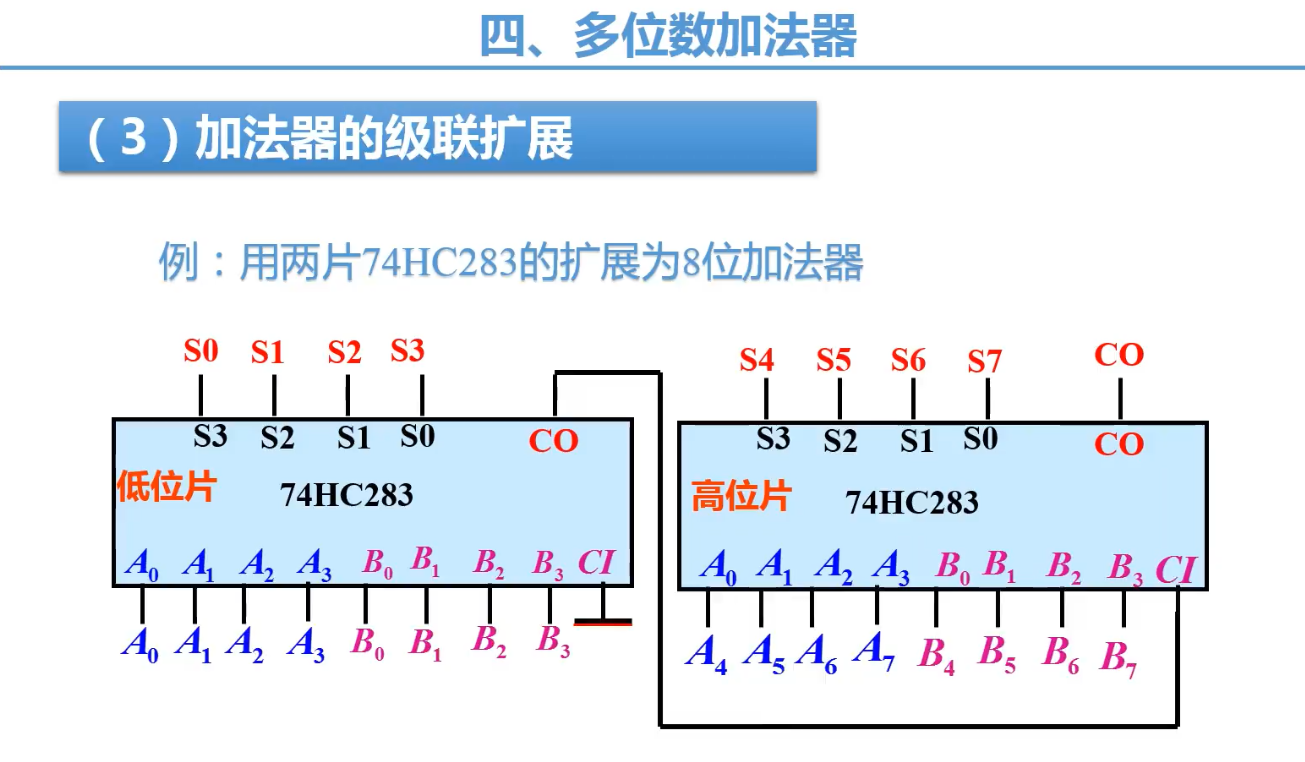后记
查看往期文章，请点击跳转 《IC前端数字验证导学》 《如何学习verilog，如何快速入门？》 《verilog学习：一个简单的入门verilog例子》 《verilog学习:使用VCS仿真验证一个全加器》 如需长期追更，可以扫码关注下面这个公众号展开全文verilog
• 二进制加法器1.1 逐位进位加法器1.2 静态CMOS加法器1.3 镜像加法器（mirror adder)1.4 传输门型加法器1.5 曼彻斯特进位链加法器1.6 进位旁路加法器(Carry-Bypass Adder)1.7 线性进位选择加法器（Linera Cary-Select...
专用集成电路 – 运算电路
@(知识点汇总)

文章目录
1.10 动态逻辑实现加法器1.10 总结
2. 乘法器2.1 阵列乘法器2.2 进位保留乘法器2.3 树形乘法器2.4 Booth乘法器
3. 移位器3.1 一位可控移位器3.2 桶形移位器3.3 对数移位器

1. 二进制加法器
二进制加法器接收加数A和B,以及进位Ci,输出和S,以及进位输出Co. 二进制加法器的真值表如下：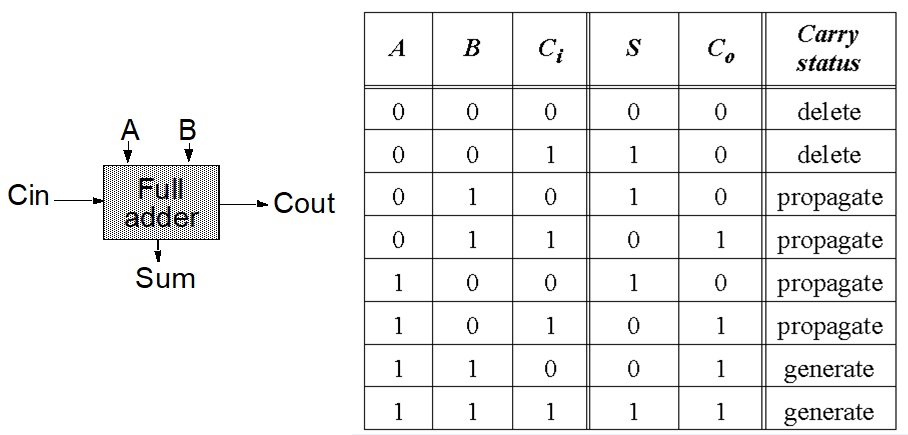逻辑表达式：

S

=

A

⊕

B

⊕

C

i

S = A \oplus B \oplus C_i

C

o

=

A

B

+

B

C

i

+

A

C

i

C_o = AB+BC_i+AC_i

从实现的角度，可以将S和Co定义为一些中间信号：

G

=

A

B

G=AB

：进位产生（generate),为1时，保证

C

o

=

1

C_o=1

D

=

A

‾

⋅

B

‾

D=\overline{A}\cdot \overline{B}

：进位取消（delet),为1时，保证

C

o

=

0

C_o=0

P

=

A

⊕

B

P = A\oplus B

：进位传播（propagate）,为1时，保证

C

o

=

C

i

C_o=C_i

上面的几个变量均和Ci无关，可以将逻辑表达式写为这些值的函数：

C

o

(

G

,

P

)

=

G

+

P

C

i

C_o(G,P) = G + PC_i

S

(

G

,

P

)

=

P

⊕

C

i

S(G,P) = P\oplus C_i

二进制全加器的一个特性是，输入反向的话，输出也反向，可以通过逻辑表达式观察得到：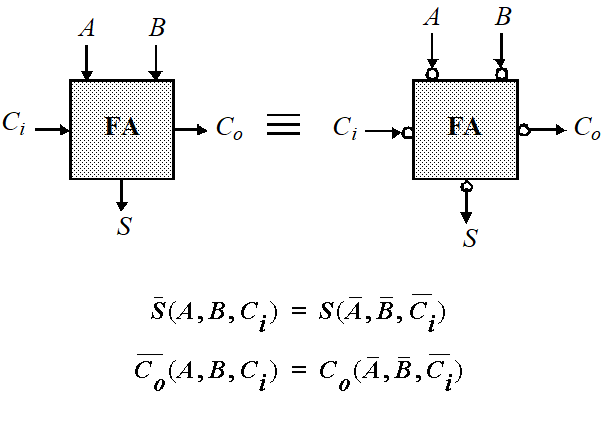1.1 逐位进位加法器
也叫行波进位加法器。通过把N个二进制全加器（FA)串联起来得到。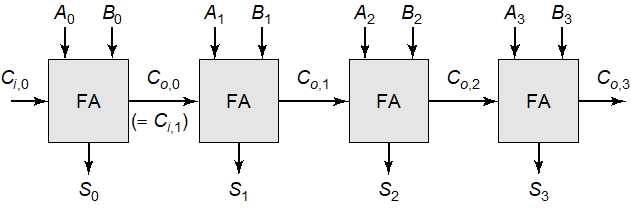对于某些输入信号，不会发生行波进位传递，而对于另一些信号，进位需要从最低有效位波动到最高位，这样一条路径称为**关键路径**（critical path),关键路径的延时定义为最坏情况下的延时。在最坏情况下的延时可以写为： $$t_{adder} = (N-1)t_{carry}+t_{sum}$$ 其中，$t_{carry}$为Ci到Co的延时，$t_{sum}$是Ci到S的延时。
1.2 静态CMOS加法器
如果直接用CMOS实现上面的二进制全加器，将会消耗36个管子，而实际上可以通过将逻辑表达式变形，来使得“和”与“进位产生”电路共享某些逻辑：

C

o

=

A

B

+

B

C

i

+

A

C

i

C_o = AB +BC_i+AC_i

S

=

A

B

C

i

+

C

o

‾

(

A

+

B

+

C

i

)

S = ABC_i+\overline{C_o}(A+B+C_i)

从真值表很容易验证这与之前的式子等价。并且只需要28管：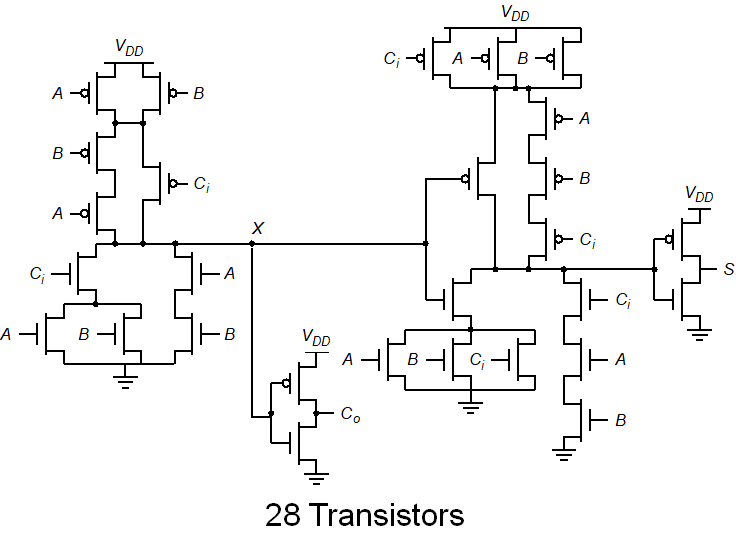上面电路用到了一个小技巧，就是将Ci关键信号放在靠近输出端，可以避免内部节点充放电等待Ci信号的到来，改善输出的延时。
缺陷：
进位产生与和产生电路中堆叠了很多PMOS管Co信号的本征负载电容很大，包括两个扩散电容，6个栅极电容加上布线电容。在进位产生电路中经过了两个反向级，如果进位链输出的负载很小，两级逻辑引起额外的延时。和的产生要求一个额外的逻辑级
延时优化： 利用全加器的反向特性，输入反向输出也反向，可以在加法器链中引入输入反向的全加器：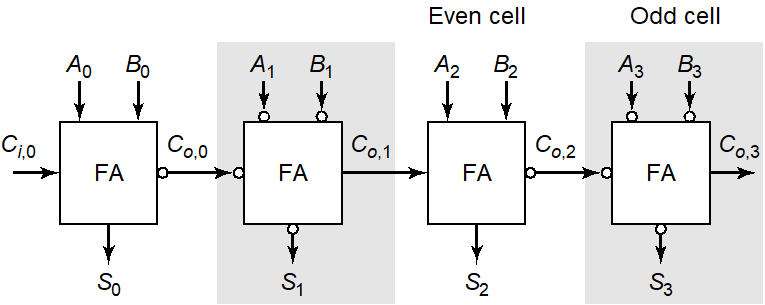图中的所有全加器的进位输出都不需要加反相器，这样大大减少了进位链的延时。
镜像加法器的特点：
基于G,D,P变量取消了进位反向门PDN和PUN不再是对偶的CMOS结构，而是对称结构。只消耗24个晶体管只有在进位产生电路的管子需要优化尺寸改善速度，求和电路的晶体管都可以使用最小尺寸。关键点在于使

C

o

‾

\overline{C_o}

处电容最小。

G

=

A

B

G=AB

，

D

=

A

‾

⋅

B

‾

D=\overline{A}\cdot \overline{B}

，$P = A\oplus B （ 与 （与 A+B$等价）

C

o

(

G

,

P

)

=

G

+

P

C

i

C_o(G,P) = G + PC_i

，

S

=

A

B

C

i

+

C

o

‾

(

A

+

B

+

C

i

)

S = ABC_i+\overline{C_o}(A+B+C_i)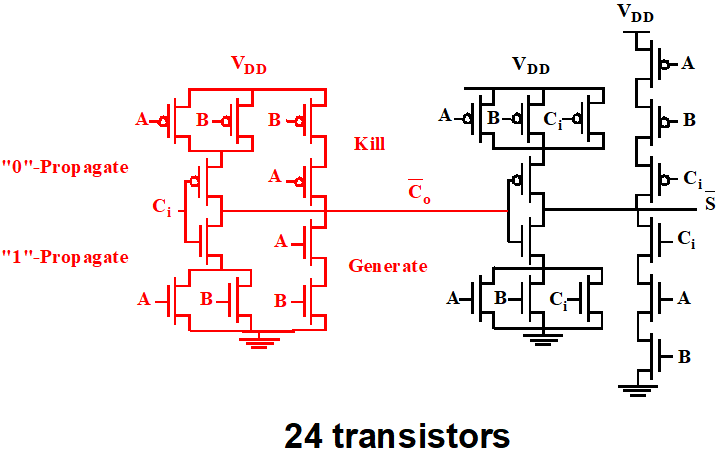可以分析一下镜像加法器的工作方式，实际上镜像加法器的进位电路巧妙利用了进位产生，消除和传播：
当A=B=1时，G=1,表示进位产生，此时通过PDN的两个串联NMOS可以将输出拉低，得到Co的反向信号，并且此时PUN不导通；当A=B=0时，D=1,表示进位消除，此时通过PUN的两个串联PMOS可以将输出拉高，得到Co的反向信号；并且此时PDN不导通；当A和B不全为1时，P=1,表示进位传播。PUN和PDN都部分导通(输入为A和B的PMOS和NMOS都有一个导通)，上拉还是下拉取决于进位输入Ci，与公式中的

P

C

i

PC_i

相匹配，并且输出Co的反向信号。对于和电路也可以同样分析。
镜像加法器的版图：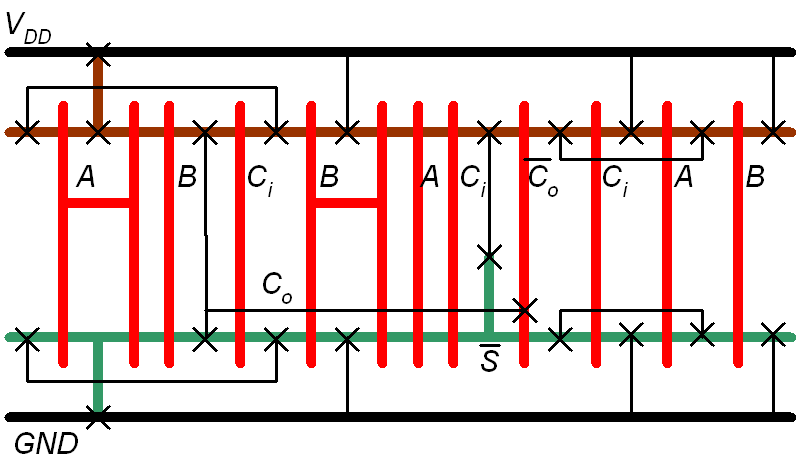1.4 传输门型加法器
使用传输门来构建和与进位电路，特点是它的和与进位输出具有近似的延时。也是24管。

G

=

A

B

G=AB

，

D

=

A

‾

⋅

B

‾

D=\overline{A}\cdot \overline{B}

，$P = A\oplus B （ 与 （与 A+B$等价）

C

o

(

G

,

P

)

=

G

+

P

C

i

C_o(G,P) = G + PC_i

，

S

(

G

,

P

)

=

P

⊕

C

i

S(G,P) = P\oplus C_i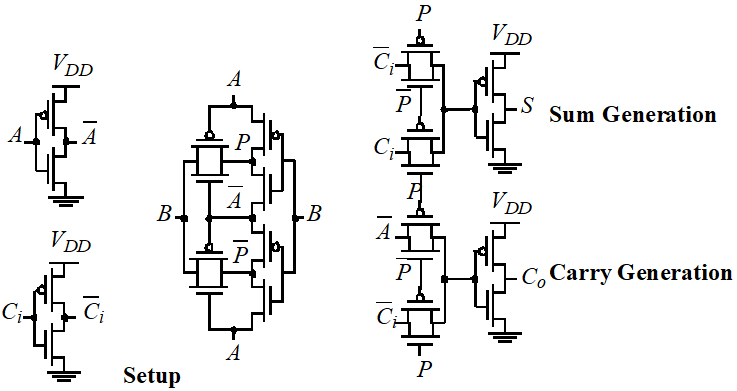需要注意的是，在Co的产生电路中，用到了一个变换：

C

o

=

P

‾

⋅

A

‾

+

P

⋅

C

i

‾

‾

=

P

C

i

+

A

C

i

+

A

P

‾

=

P

C

i

+

A

⋅

A

⊙

B

=

P

C

i

+

A

B

C_o=\overline{\overline{P} \cdot \overline{A} + P\cdot \overline{C_i}} = PC_i+AC_i+A\overline{P}=PC_i+A\cdot A\odot B = PC_i+AB

1.5 曼彻斯特进位链加法器
在传输门加法器中，进位电路可以进行优化，比如可以使用进位消除和产生信号来控制进位电路（下左图）：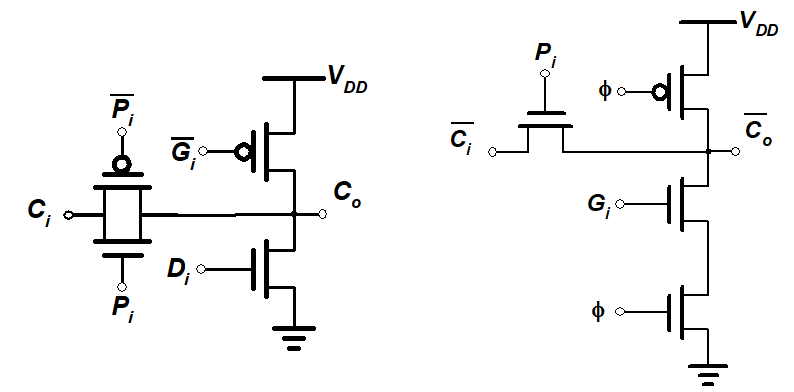此外，使用动态逻辑可以更加简化，传输门也只需要使用单管，并且不需要进位取消电路。如上面右图。在预充电（

ϕ

=

0

\phi=0

)时，输出节点充到VDD，表示Co=0;求值时，根据G和Ci信号决定有无进位产生。下图是曼彻斯特进位链的构造：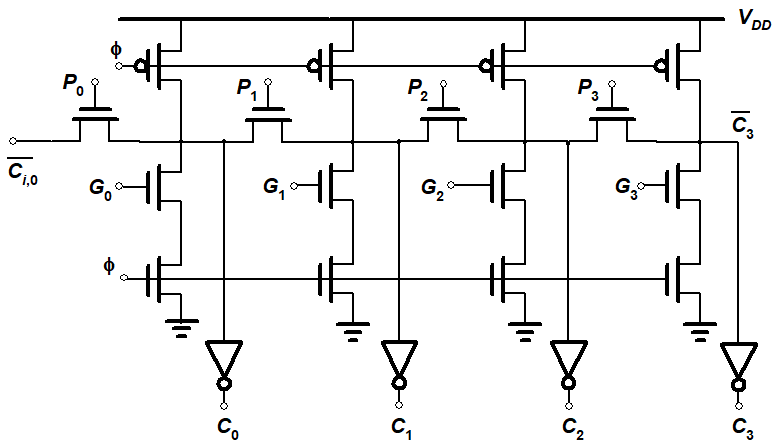上图中加法器进位链在最坏情况下的延时模型可模拟为线性RC网络。其延时为：

t

p

=

0.69

∑

i

=

1

N

C

i

(

∑

j

=

1

i

R

j

)

=

0.69

N

(

N

+

1

)

2

R

C

t_p = 0.69\sum_{i=1}^N C_i(\sum_{j=1}^iR_j) = 0.69\frac{N(N+1)}{2}RC

版图：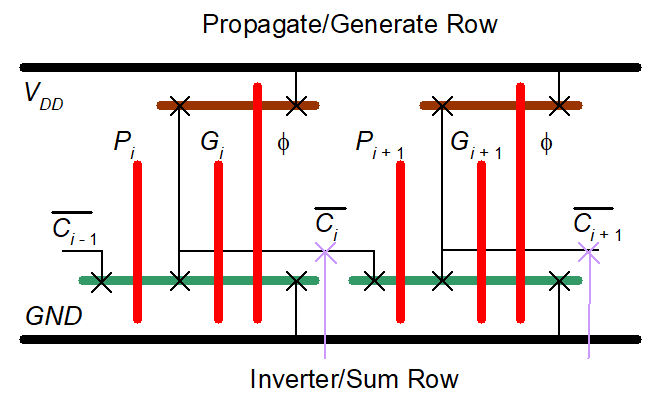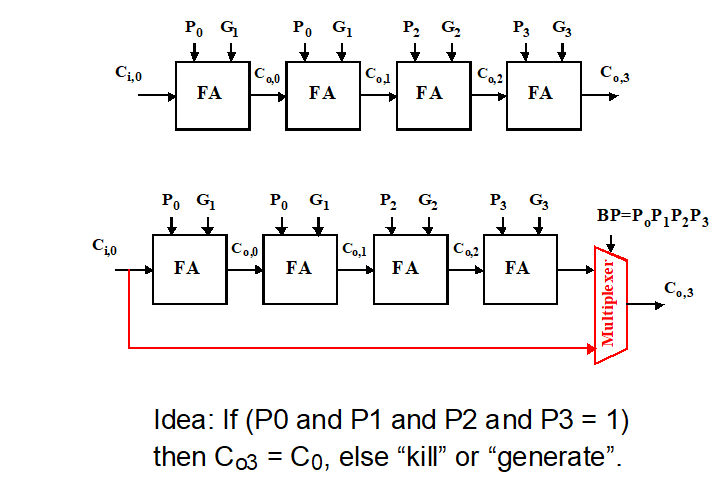基本思路是增加一条进位旁路，通过进位选择信号

B

P

=

P

0

P

1

…

P

n

BP=P_0P_1\dots P_n

信号来选择进位输出是否直接选择最底层的进位进行输出。进而加快加法器。
曼彻斯特进位链实现的旁路加法器：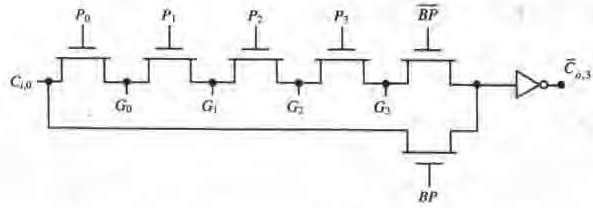从上图中可以发现，当BP为高时，直接输出进位输入，而BP不为高时，则输出由中间的某些G信号决定，与Ci无关。这一性质可以极大加速加法器链，因为若干个这样的模块串联在一起时，实际上各个模块的进位电路中直到选择器之前的部分都可以并行地执行，而无需等待上级进位信号。
下面来计算旁路加法器的传播延时。首先对于N级的旁路进位加法器，假设其被划分为（N/M）个等长的旁路，每个旁路含有M位：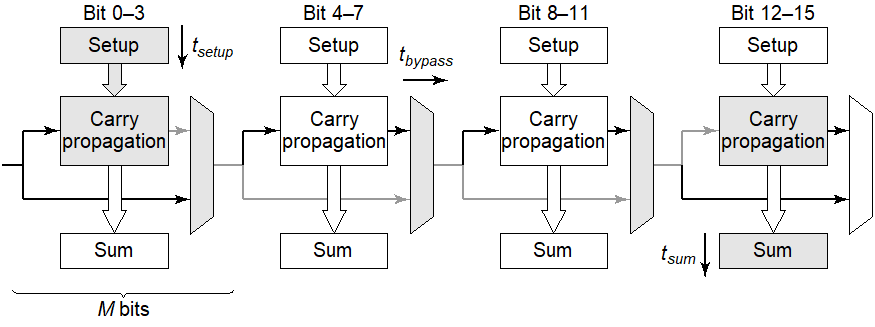上图中灰色部分为关键路径。其延时可以表示为：

t

p

=

t

s

e

t

u

p

+

M

t

c

a

r

r

y

+

(

N

M

−

1

)

t

b

y

p

a

s

s

+

(

M

−

1

)

t

c

a

r

r

y

+

t

s

u

m

t_p = t_{setup} + Mt_{carry}+(\frac{N}{M}-1)t_{bypass}+(M-1)t_{carry}+t_{sum}

可以看到，虽然旁路加法器的延时仍然关于N呈线性关系，但斜率除以了一个M，但是多了几个常数项，所以反映到下图的延时和级数N的关系曲线中就会发现，当N比较大时旁路加法器优于逐位进位，但N比较小（4-8）时，反而比逐位进位差。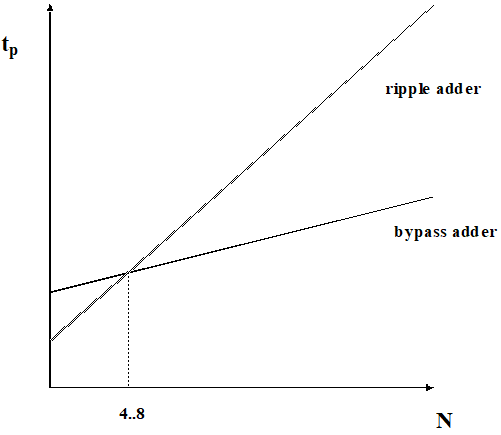线性进位选择加法器其实跟上面的旁路加法器类似，但是旁路加法器可以工作的一个条件是进位链中要使用G信号或者D信号来保证不选择旁路时加法器链的输出也跟输入进位Ci无关。因此旁路加法器需要额外的BP信号（每级P相与）配合进位产生信号G来控制进位链。 另外一种方法是使用线性进位选择加法器，其工作更加简单粗暴，为了使得加法器链的进位输出跟输入解耦，直接考虑进位输入的两种可能值，提前计算两种可能结果，等到输入进位到来直接根据进位值选择哪条路径输出即可，这意味着同时实现了0和1进位输入的两个进位路径：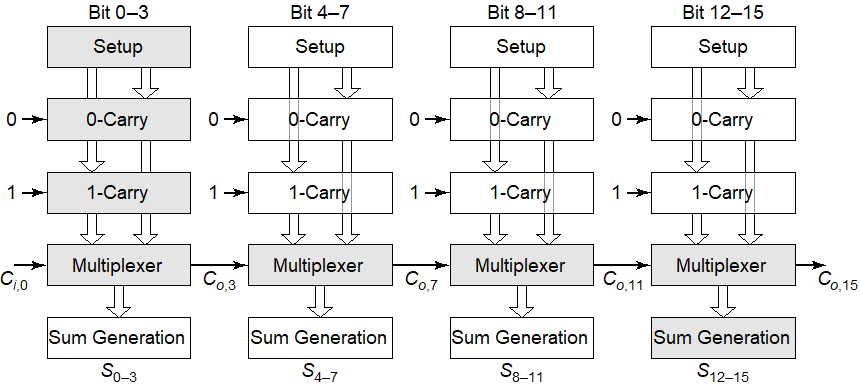付出的代价是一个额外的进位路径和一个多路开关，大概等于逐位进位结构的30%。 可以推导进位选择加法器的传播延时为：

t

p

=

t

s

e

t

u

p

+

M

t

c

a

r

r

y

+

(

N

M

)

t

m

u

x

+

t

s

u

m

t_p = t_{setup}+Mt_{carry}+(\frac{N}{M})t_{mux}+t_{sum}

可见其传播延时仍然关于N呈线性关系。
在上面的线性选择加法器中，每级分配相同的全加器，因此每级的延时都相同，每级进位链的结果在上一级的多路开关信号到来前已经稳定下来，可见在后面的几级在时刻5已经完成，但每级等待的时间越来越长，如图：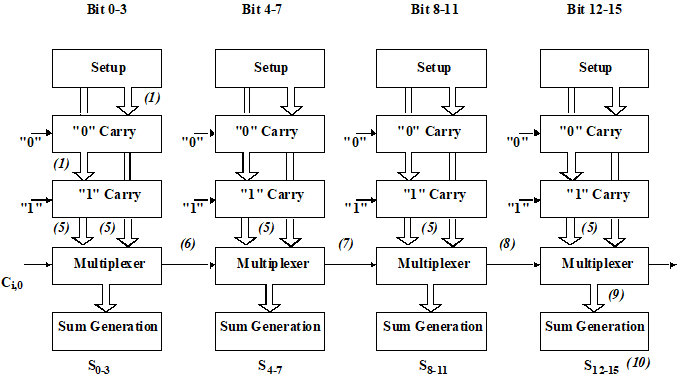如果能够使后级不需要等待前一级多路开关的信号，而是在刚求完本级就正好接受到前级信号，就能消除这个等待时间：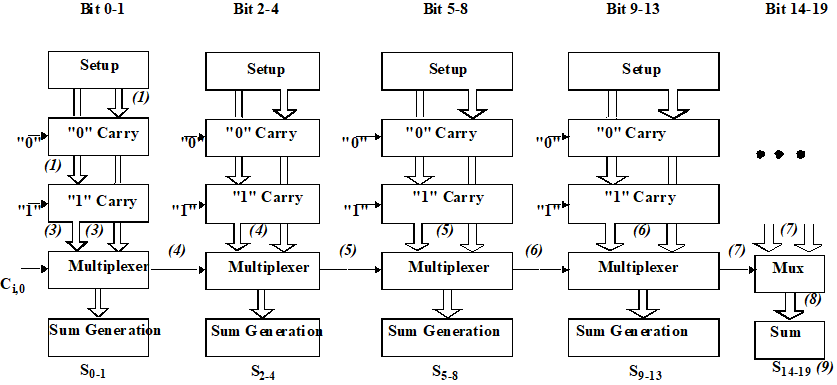上图就是平方根进位选择加法器，其与线性选择加法器的区别只在于每级的位数逐级递增。通过这种方式，每级在计算完本级的进位结果的同时上一级的多路开关信号正好到来。 假设总共含有

P

P

级，第一级为

M

M

位。则有

N

=

M

+

(

M

+

1

)

+

(

M

+

2

)

+

⋯

+

(

M

+

P

−

1

)

=

p

2

2

+

P

(

M

−

1

2

)

N = M + (M+1)+(M+2)+\dots+(M+P-1)=\frac{p^2}{2}+P(M-\frac{1}{2})

如果

M

<

<

N

,

如

M

=

2

,

N

=

64

M<<N,如M=2,N=64

,则第一项起主要作用，则有

N

=

P

2

2

,

P

=

2

N

N = \frac{P^2}{2}, P=\sqrt{2N}

因此其传播延时可写为：

t

p

=

t

s

e

t

u

p

+

M

t

c

a

r

r

y

+

(

2

N

)

t

m

u

x

+

t

s

u

m

t_p = t_{setup}+Mt_{carry}+(\sqrt{2N})t_{mux}+t_{sum}

从公式可以看到，由于此处的M是初级的位数，一般都比线性选择加法器的小，延时并且跟根号2N呈线性关系，所以反应到延时和级数曲线中，平方根进位选择加法器跟纵轴交点要小于线性选择，且当N比较大时斜率远小于线性选择加法器：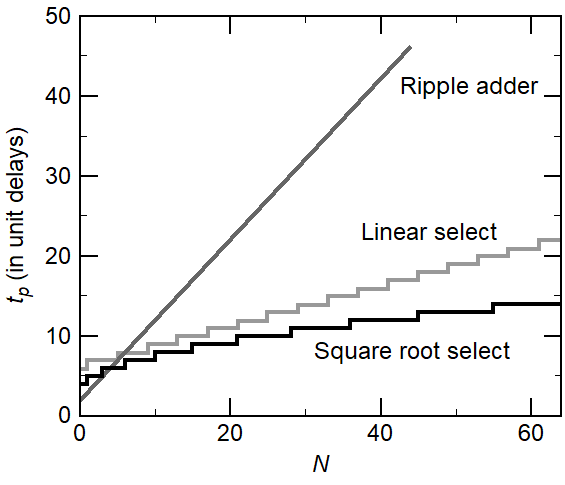a. 单一超前进位加法器
上面的选择和旁路加法器虽然能减少延时，但逐级进位效应仍然存在。超前进位加法器解决了这一问题。 其基本思想是： N位加法器的每一位上都有下面的关系：

C

o

,

k

=

f

(

A

k

,

B

k

,

C

o

,

k

−

1

)

=

G

k

+

P

k

C

o

,

k

−

1

C_{o,k}=f(A_k,B_k,C_{o,k-1})=G_k+P_kC_{o,k-1}

通过展开

C

o

,

k

−

1

C_{o,k-1}

，一直展开到第一级可以彻底消除每级进位的前一级的依赖，代价是实现加法器的逻辑逐级增加：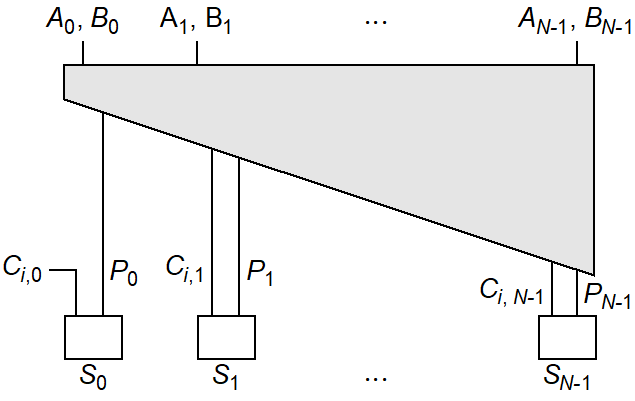下面是一个四级超前进位加法的实现，利用了对偶性（类似镜像加法器）：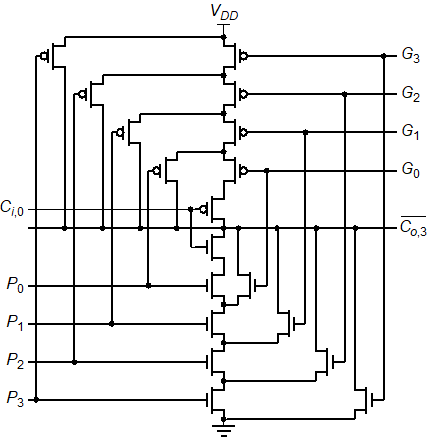局限： 单一超前进位加法器只在N比较小（<5)时使用，实际上的延时还是随位数线性增加的，这主要由下面的因素决定：
大的扇入使得在N较大时极慢如果用较为简单的门实现，则需要多个逻辑层次，也会增加延时某些信号的扇出常常过度增加，使得加法器更慢,例如G0和P0信号出现在后面的每级中，导致线电容较大
b. 对数超前进位加法器
将进位传播和进位产生组织成递归的树状结构：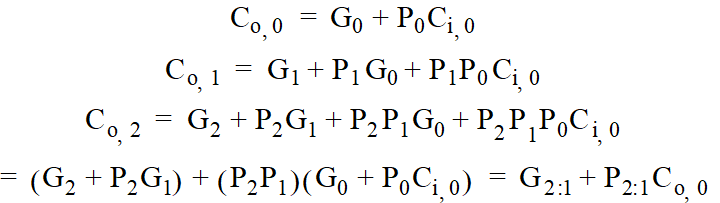在上面的公式中，进位传播被分解成两位的子组合

G

i

:

j

,

P

i

:

j

G_{i:j},P_{i:j}

.称之为块进位产生和块进位传播信号。 引入一个新的布尔运算，称之为点操作

(

⋅

)

(\cdot)

：

(

G

,

P

)

⋅

(

G

′

,

P

′

)

=

(

G

+

P

G

′

,

P

P

′

)

(G,P)\cdot (G',P')=(G+PG',PP')

利用这个操作可以分解

(

G

i

:

j

,

P

i

:

j

)

=

(

G

i

:

k

,

P

i

:

k

)

⋅

(

G

k

−

1

:

j

,

P

k

−

1

:

j

)

(G_{i:j},P_{i:j})=(G_{i:k},P_{i:k})\cdot (G_{k-1:j},P_{k-1:j})

,点操作服从结合律，不服从交换律。 利用点操作的结合律，可以构成一个树结构来计算所有

2

i

−

1

2^i-1

个位置上的进位，并且只需要

l

o

g

2

(

N

)

log_2(N)

就可计算出来。因此对数加法器可以将传播延时降低到

l

o

g

2

(

N

)

log_2(N)

。 下面是基于点操作的基2 kogge-Stone草签仅为对数加法器原理图。 其中白色小方格表示建立P和G信号，黑点表示点操作，菱形表示和产生。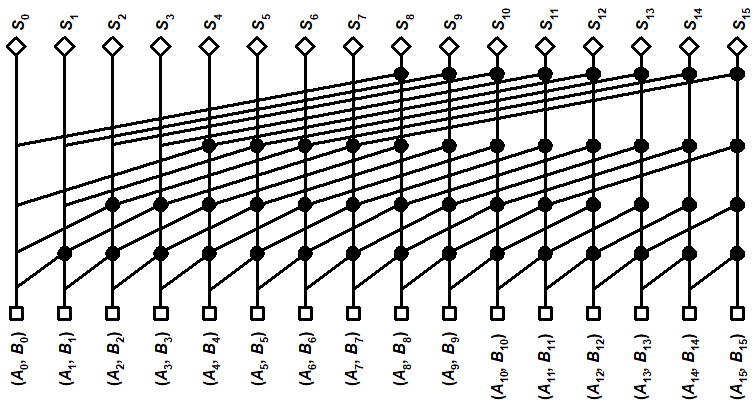对于N=16的情形，整个加法器需要49个逻辑门。
1.10 动态逻辑实现加法器
下面是用动态多米诺逻辑实现的加法器进位产生和传播信号电路。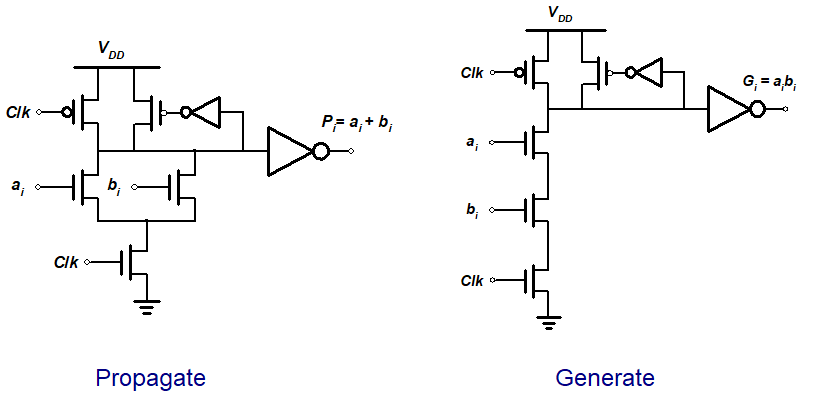以及多米诺逻辑实现的点操作电路：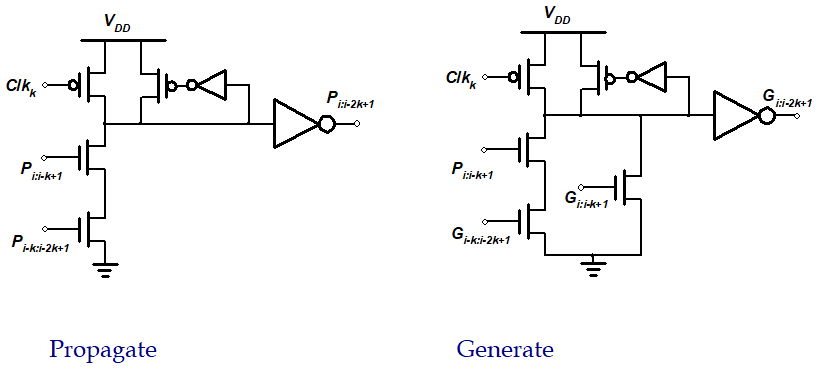还需要配套的求和电路：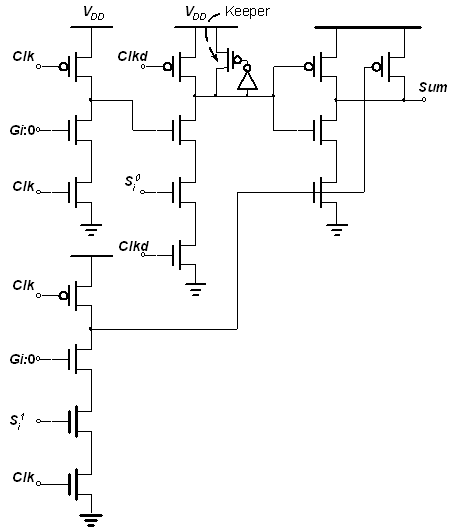其中

S

i

0

=

a

i

⊕

b

i

‾

S_i^0=\overline{a_i\oplus b_i}

,

S

i

1

=

a

i

⊕

b

i

S_i^1=a_i\oplus b_i

.上图中的保持器是重要的，因为前面两级多米诺逻辑违反了多米诺的设计规则：两个动态门串联在一起而没有在中间插入反相器。如果用同一个时钟求值，则会第二个门的输出可能会有毛刺。
1.10 总结
各类加法器传播延时和面积与级数的关系图：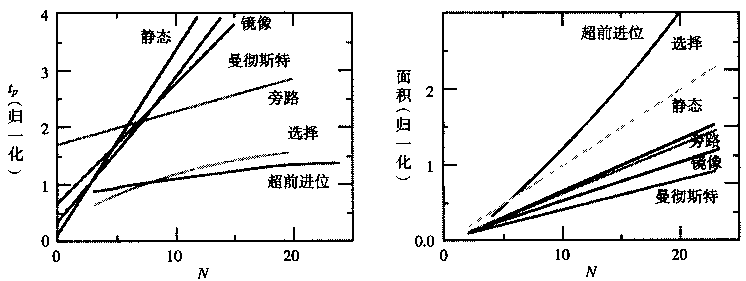2. 乘法器
2.1 阵列乘法器
实现乘法的比较常用的方法是类似与手工计算乘法的方式：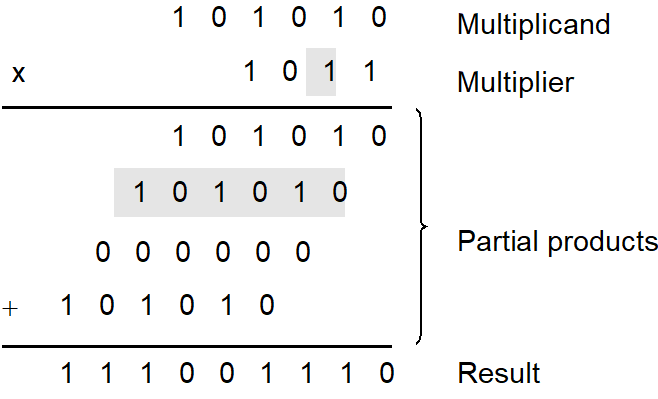对应的硬件结构就是阵列乘法器（array multiplier）它有三个功能：产生部分积，累加部分积和最终相加。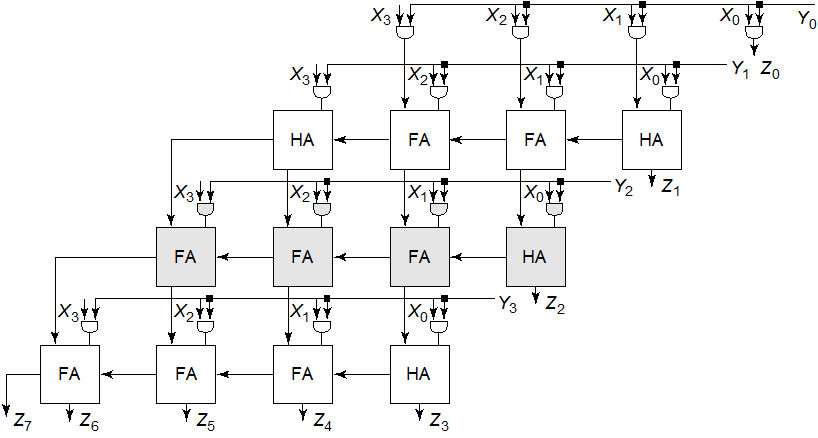阵列乘法器的关键路径为(下图标出了两条可能的关键路径）：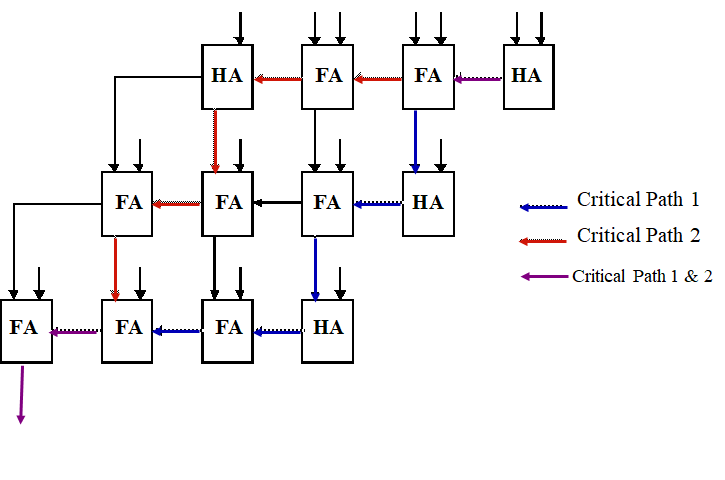假设乘数为

N

N

,被乘数为

M

M

。则上面的乘法器产生

N

N

个部分积，乘法位数为

M

M

,则需要

N

×

M

N\times M

个二输入AND门以及

N

−

1

N-1

个

M

M

位加法器。 可以得到关键路径延时为：

t

m

u

l

t

=

[

(

M

−

1

)

+

(

N

−

2

)

]

t

c

a

r

r

y

+

(

N

−

1

)

t

s

u

m

+

t

a

n

d

t_{mult} = [(M-1)+(N-2)]t_{carry}+(N-1)t_{sum}+t_{and}

2.2 进位保留乘法器
背景：由于阵列乘法器中有许多几乎一样的关键路径，因此通过调整晶体管的尺寸来提高性能效果有限。
解决：进位输出可以沿着对角线传播而不是向左传递，这样传播并不改变结果，只需要加入额外的一排加法器（向量合并加法器vector-merging)来产生最终的结果。这样的乘法器叫做进位保留乘法器：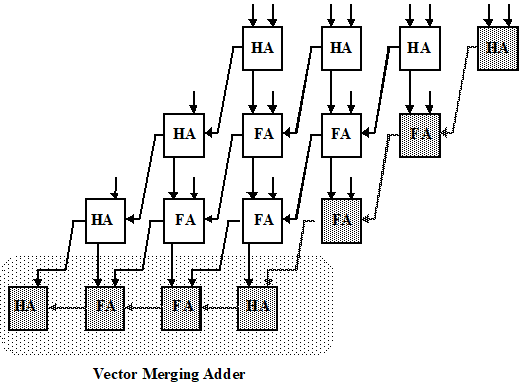其关键路径为图中灰色部分，且只有一条。 其传播延时为： $$t_{mult} = t_{and} + (N-1)t_{carry}+t_{merge}$$
可见，相比于阵列乘法器，进位保留乘法器增加了部分面积，但关键路径唯一确定。
下图是进位保留加法器的布图（floorplan)结构。其规整的结构适合自动化生成。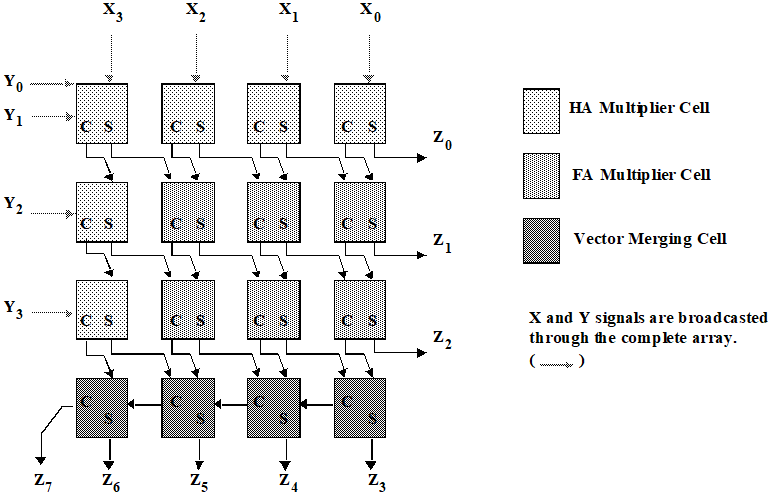2.3 树形乘法器
部分积的求和加法器可以安排为树形以减少关键路径和减少加法器。其中两个点的是半加器，三个点的是全加器。其压缩过程可以分为下面几步：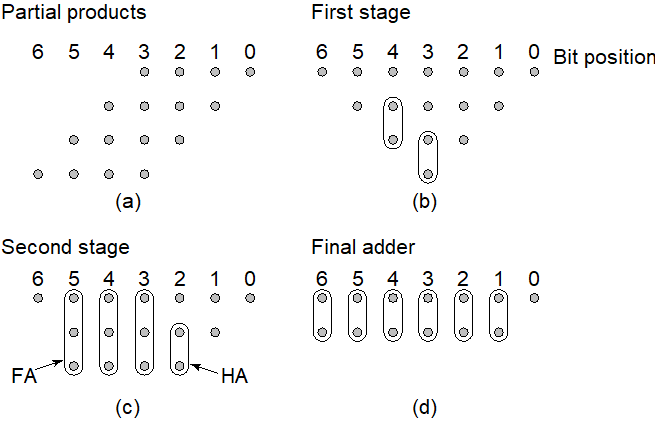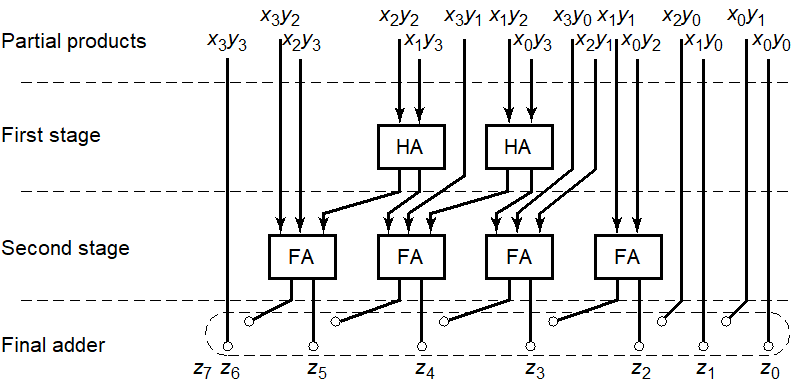可见，前面的两部只需要三个半加器和三个全加器，最后一步是简单的加法器链。只比较前面部分的话，进位保留加法器的前面部分需要6个全加器和6个半加器！ 优点：除了节省乘法器需要的硬件，Wallace树形乘法器也可以减少传播延时，其延时

O

(

l

o

g

1.5

(

N

)

)

O(log_{1.5}(N))

。
缺点： 非常不规则，版图设计复杂。
2.4 Booth乘法器
在下面详细说明了Booth乘法器原理：

https://www.cnblogs.com/lyc-seu/p/12842399.html

3. 移位器
3.1 一位可控移位器
其实是一个复杂的多路开关电路，根据不同控制信号，将输入左移或右移或不变。 多位的移位可以简单串联这样的单元实现，但移位位数多时，该方法过于复杂，不实用并且速度很慢。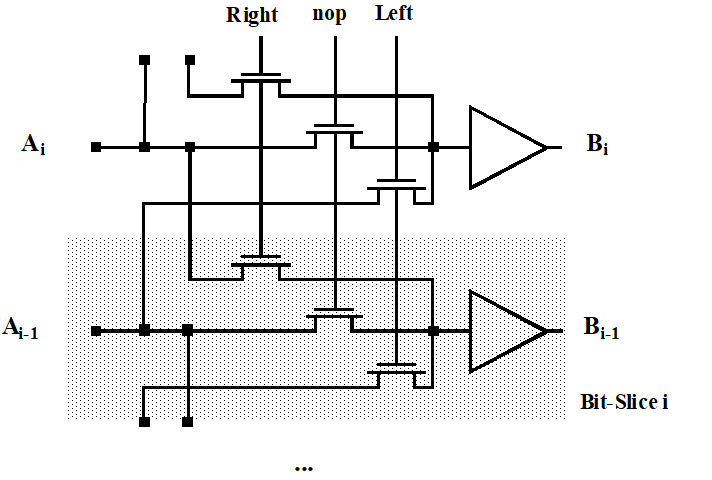3.2 桶形移位器
由晶体管阵列构成，行数等于数据字长，列数等于最大移位宽度。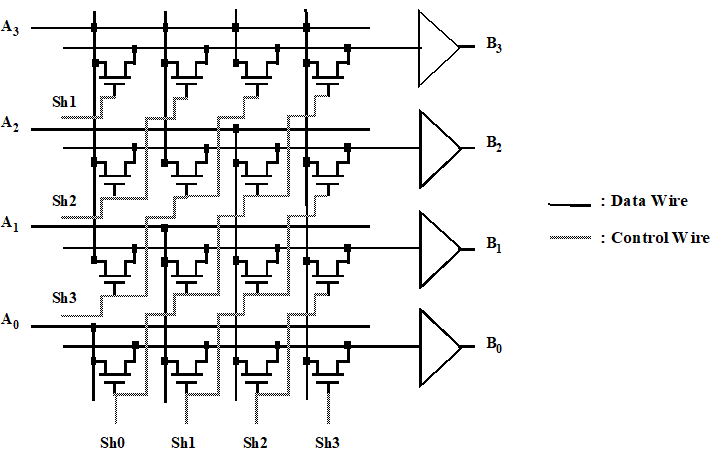特点：
Sh0Sh3**只能有一位是高电平**，分别控制A右移0位3位自动支持符号位扩展信号最多只需要通过一个传输门，也就是说传播延时理论上是常数，与位数和规模无关（实际上因为缓冲器输入电容会随着移位宽度线性增加，所以延时不会是常数）版图尺寸不由晶体管决定，而是通过布线数目或者所金属线的间距决定。适用于移位数较小的移位器。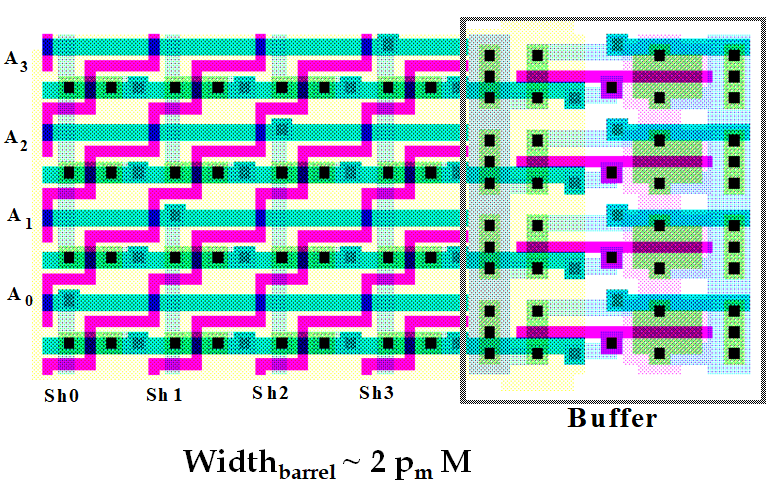3.3 对数移位器
桶形移位器实现为传输管的单个阵列，而对数移位器采用分级的方法。移位值分解为几个2的指数值。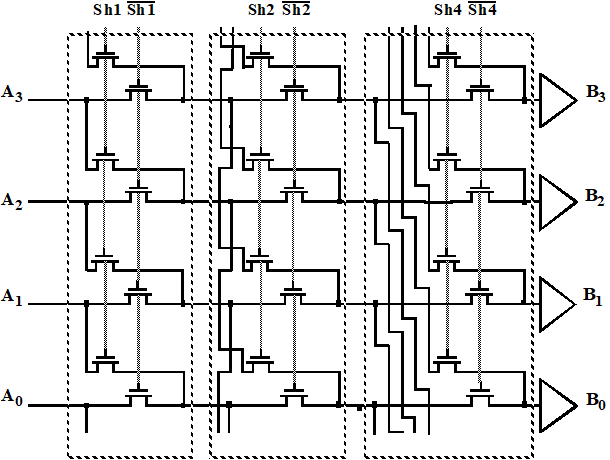对数移位器的速度取决于移位宽度，一个M为的移位器需要

l

o

g

2

M

log_2M

级，适用于较大的移位值，在速度和面积上都更有效，且容易参数化。
版图：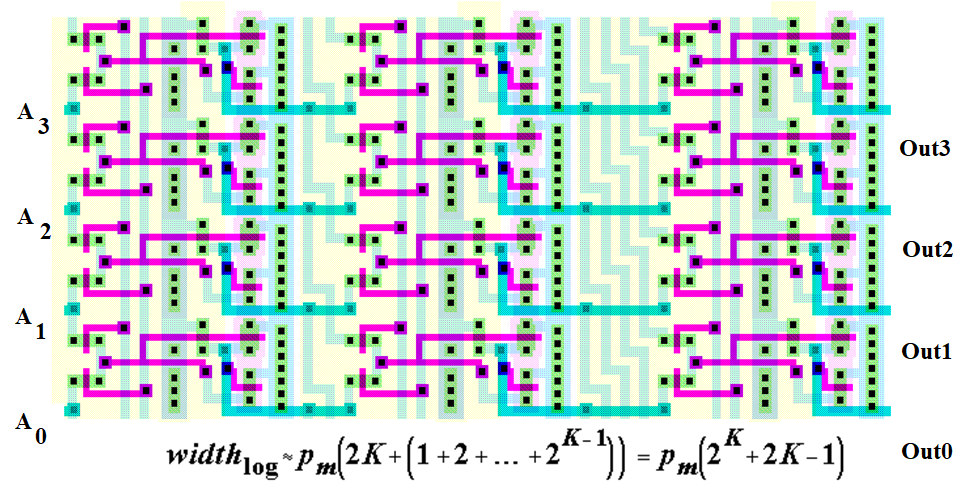展开全文• 数电书上说道超前进位加法器，没有仔细讲。上网搜了这篇资料，摘抄下来 串行进位加法器需要一级一级的进位，进位延迟很大。先行进位加法器（也叫超前进位加法器）可以有效的减少进位延迟。 设二进制加法器的第i...
• 如果对传统的加法器电路进行改进,在超前进位链的基础上,用一种新的超前进位链树的设计方法不仅可以克服串行进位加法器速度低的缺点,也可以解决单纯的超前进位加法器带负载能力不足等问题,从而在实际电路中使加法器的...
• 先行进位加法器是对普通的全加器进行改良而设计成的并行加法器，主要是针对普通全加器串联时互相进位产生的延迟进行了改良。超前进位加法器是通过增加了一个不是十分复杂的逻辑电路来做到这点的。 设二进制加法器第i...
• 1074 宇宙无敌加法器 （20 分） 地球人习惯使用十进制数，并且默认一个数字的每一位都是十进制的。而在 PAT 星人开挂的世界里，每个数字的每一位都是不同进制的，这种神奇的数字称为“PAT数”。每个 PAT 星人都必须...C PAT
• AxMAP探索设计空间并使用随机搜索算法生成近似加法器。 在每次迭代中，满足约束条件的所选设计均被视为有效输出。 有关更多详细信息，请阅读有关AxMAP的文章。 设置和使用AxMAP 要求 该代码已在装有Corei5 2.5GHz...
• 本文提供了一个同步清零、同步置数的十进制加法计数器代码和一个异步清零、异步置数的可逆十进制计数器代码，且使用ISE13.4综合通过并在Basys2开发板上成功验证功能，此外大家可以修改代码以调节周期。同步清零、...Verilog FPGA
• `timescale 1ns / 1ps//////////////////////////////////////////////////////////////////////////////////// 超前进位加法器，并行加法计算///////////////////////////////////////////////////////////////////...
• 在线性方程中，一个限制条件可以减少方程中一个因变量。那么试想，如果没有把这个限制条件提前消耗在以计算方便为目的假设中，我们最后计算应该得到的是两个未确定的因变量的关系式。我们就可以根据实际情况自由选择...multism 运放
• 一、移位相加乘法—串行形式 1、RTL代码 module unsigned_mul_1 #( parameter DATAWIDTH=8 )(clk, x, y, result); parameter s0 = 0, s1 = 1, s2 = 2; input clk; input [DATAWIDTH-1:0] x, y; output ...verilog fpga 算法
• 计算机所做的计算处理只有加法，有了加法就可以用加法计算除法，乘法，减法。而计算机所处理的数据也只是二进制数也就是0和1。下面简单阐述二进制加法机的构造原理，这是cpu计算单元的基本计算原理。 加法计算实际上...cpu
• 加法：  整数： [A]补 + [B]补 = [A+B]补 （mod 2^(n+1)）  小数： [A]补 + [B]补 = [A+B]补 （mod 2） 减法：  整数： [A-B]补 = [A]补 + [-B]补 （mod 2^(n+1)）  小数： [A-B]补 = [A]补 + [-B]补（mod 2）...ZYNQ SDK Vivado HLS 硬件设计
• 来源：21IC中国电子网 / 作者：夏有为 林正浩 杨晓峰 发表时间：2006-04-02 从CPU的指令执行频率上看，算术逻辑单元、程序计数器、协处理器是CPU中使用频率最多的模块，而加法器正是这些模块的核心部件，几乎所有...嵌入式 c 算法
• 那么，仅仅利用上一篇博文实现的变量加法器作为地基去把复杂的算法搭建起来还是有点天方夜谭，还缺少很多必要的元素，其中就包括指针（数组）的使用。比如我们之后要实现的 FFT算法 ，其输入输出都是一个长数组，...ZYNQ SDK HLS Vivado 硬件设计
•  在给定训练数据及损失函数的条件下，学习加法模型成为经验风险极小化问题，即损失函数极小化问题：  随后，该问题可以作如此简化：从前后，每一步只学习一个基函数及其系数，逐步逼近上式，即：每...
• 由于后面需要使用综合工具进行综合，这里先声明使用的FPGA是Virtex-7系列的： 目的很单纯，就是熟悉一下使用ISE进行FPGA设计的一般流程。 硬件语言描述 首先使用行为级描述方式的硬件描述语言（HDL）设计一个一...
• 提出一种针对乘法的低功耗设计算法,其优化指标为乘法内部加法运算次数.在实现技术上,解决了目前低功耗设计中算法自身逻辑单元被引入系统从而降低系统优化效果的问题.该算法能够在不降低系统工作效率、不损失系统...神经网络 人工智能
• 2 学习加法模型的前分步算法步骤如下： 三 adaboost 1 定理： 2 解析： 3 证明：  (1)基函数  (2)损失函数 四 代码实现   五 总结 一 加法模型 1 什么是加法模型 加法模型(additive model)又叫可加...
• 对于数在计算机里的加减法，由于有了补码这个编码之后，其在计算机里的加减法就变得容易许多，毕竟减法用补码处理的时候可以转化为加法解决，这也是为什么不直接用原码处理加减法的一个原因，在这里分别对这两个基础...
• 算术和逻辑指令 ADC : 带进位的加法 (Addition with Carry) ADC{条件}{S} , , ... dest = op_1 + op_2 +...它使用一个进位标志位，这样就可以做比 32 位大的加法。下列例子将加两个 128 位的数。 128 位结果: 寄arm处理器 ARM指令集
•向量代数 应用...